: 330-486-0706
sales@rfiamericas.com

# Antenna Theory – Fundamentals

Home » News » Antenna Theory » Antenna Theory – Fundamentals

A person, who needs to convey a thought, an idea or a doubt, can do so by voice communication.

The following illustration shows two individuals communicating with each other. Here, communication takes place through sound waves. However, if two people want to communicate who are at longer distances, then we have to convert these sound waves into electromagnetic waves. The device, which converts the required information signal into electromagnetic waves, is known as an Antenna.

## What is an Antenna ?

An Antenna is a transducer, which converts electrical power into electromagnetic waves and vice versa.

An Antenna can be used either as a transmitting antenna or a receiving antenna.

• transmitting antenna is one, which converts electrical signals into electromagnetic waves and radiates them.
• receiving antenna is one, which converts electromagnetic waves from the received beam into electrical signals.
• In two-way communication, the same antenna can be used for both transmission and reception.

Antenna can also be termed as an Aerial. Plural of it is, antennae or antennas. Now-adays, antennas have undergone many changes, in accordance with their size and shape. There are many types of antennas depending upon their wide variety of applications.

Following pictures are examples of different types of Antennas.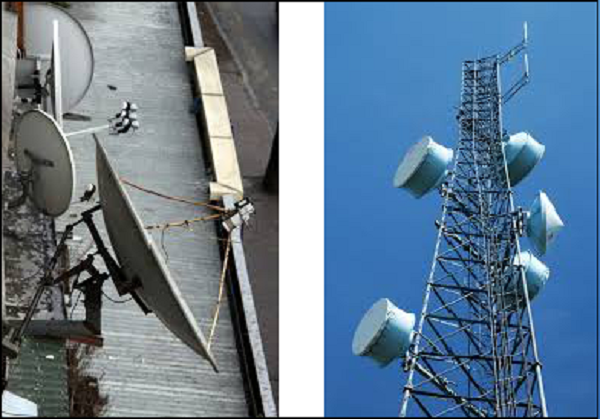In this chapter, you are going to learn the basic concepts of antenna, specifications and different types of antennas.

## Need of Antenna

In the field of communication systems, whenever the need for wireless communication arises, there occurs the necessity of an antenna. Antenna has the capability of sending or receiving the electromagnetic waves for the sake of communication, where you cannot expect to lay down a wiring system. The following scenario explains this.

### Scenario

In order to contact a remote area, the wiring has to be laid down throughout the whole route along the valleys, the mountains, the tedious paths, the tunnels etc., to reach the remote location. The evolution of wireless technology has made this whole process very simple. Antenna is the key element of this wireless technology.In the above image, the antennas help the communication to be established in the whole area, including the valleys and mountains. This process would obviously be easier than laying a wiring system throughout the area.

The sole functionality of an antenna is power radiation or reception. Antenna (whether it transmits or receives or does both) can be connected to the circuitry at the station through a transmission line. The functioning of an antenna depends upon the radiation mechanism of a transmission line.

A conductor, which is designed to carry current over large distances with minimum losses, is termed as a transmission line. For example, a wire, which is connected to an antenna. A transmission line conducting current with uniform velocity, and the line being a straight one with infinite extent, radiates no power.

For a transmission line, to become a waveguide or to radiate power, has to be processed as such.

• If the power has to be radiated, though the current conduction is with uniform velocity, the wire or transmission line should be bent, truncated or terminated.
• If this transmission line has current, which accelerates or decelerates with a time varying constant, then it radiates the power even though the wire is straight.
• The device or tube, if bent or terminated to radiate energy, then it is called as waveguide. These are especially used for the microwave transmission or reception.

This can be well understood by observing the following diagram −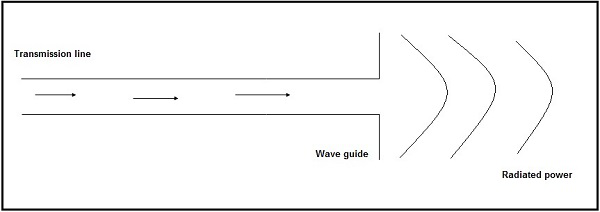The above diagram represents a waveguide, which acts as an antenna. The power from the transmission line travels through the waveguide which has an aperture, to radiate the energy.

## Basic Types of Antennas

Antennas may be divided into various types depending upon −

• The physical structure of the antenna.
• The frequency ranges of operation.
• The mode of applications etc.

### Physical structure

Following are the types of antennas according to the physical structure. You will learn about these antennas in later chapters.

• Wire antennas
• Aperture antennas
• Reflector antennas
• Lens antennas
• Micro strip antennas
• Array antennas

### Frequency of operation

Following are the types of antennas according to the frequency of operation.

• Very Low Frequency (VLF)
• Low Frequency (LF)
• Medium Frequency (MF)
• High Frequency (HF)
• Very High Frequency (VHF)
• Ultra High Frequency (UHF)
• Super High Frequency (SHF)
• Micro wave

### Mode of Applications

Following are the types of antennas according to the modes of applications −

• Point-to-point communications
• Satellite communications

# Antenna Theory – Basic Parameters

The basic communication parameters are discussed in this chapter to have a better idea about the wireless communication using antennas. The wireless communication is done in the form of waves. Hence, we need to have a look at the properties of waves in the communications.

In this chapter, we are going to discuss about the following parameters −

• Frequency
• Wavelength
• Impedance matching
• VSWR & reflected power
• Bandwidth
• Percentage bandwidth

Now, let us learn them in detail.

## Frequency

According to the standard definition, “The rate of repetition of a wave over a particular period of time, is called as frequency.”

Simply, frequency refers to the process of how often an event occurs. A periodic wave repeats itself after every ‘T’ seconds (time period). Frequency of periodic wave is nothing but the reciprocal of time period (T).

### Mathematical Expression

Mathematically, it is written as shown below.

f=1Tf=1T

Where

• f is the frequency of periodic wave.
• T is the time period at which the wave repeats.

### Units

The unit of frequency is Hertz, abbreviated as Hz.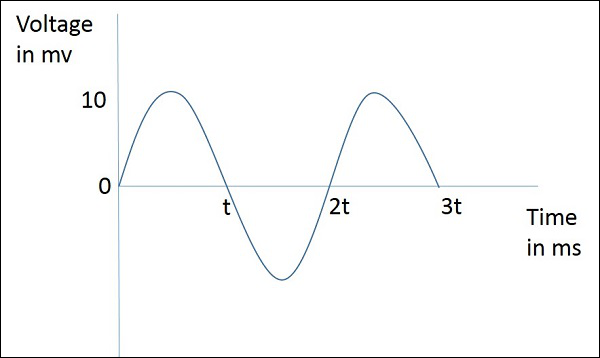The figure given above represents a sine wave, which is plotted here for Voltage in millivolts against time in milliseconds. This wave repeats after every 2t milliseconds. So, time period, T=2t milliseconds and frequency, f=12TKHzf=12TKHz

## Wavelength

According to the standard definition, “The distance between two consecutive maximum points (crests) or between two consecutive minimum points (troughs) is known as the wavelength.”

Simply, the distance between two immediate positive peaks or two immediate negative peaks is nothing but the length of that wave. It can be termed as the Wavelength.

The following figure shows a periodic waveform. The wavelength (λ) and amplitude are denoted in the figure. The higher the frequency, the lesser will be the wavelength and vice versa.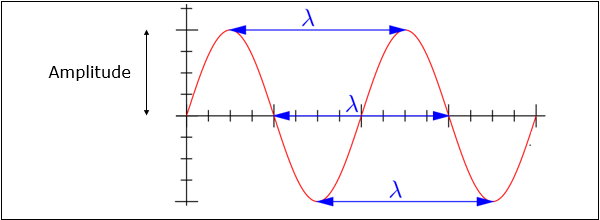### Mathematical Expression

The formula for wavelength is,

λ=cfλ=cf

Where

• λ is the wavelength
• c is the speed of light (31083∗108 meters/second)
• f is the frequency

### Units

The wavelength λ is expressed in the units of length such as meters, feet or inches. The commonly used term is meters.

## Impedance Matching

According to the standard definition, “The approximate value of impedance of a transmitter, when equals the approximate value of the impedance of a receiver, or vice versa, it is termed as Impedance matching.”

Impedance matching is necessary between the antenna and the circuitry. The impedance of the antenna, the transmission line, and the circuitry should match so that maximum power transfer takes place between the antenna and the receiver or the transmitter.

### Necessity of Matching

A resonant device is one, which gives better output at certain narrow band of frequencies. Antennas are such resonant devices whose impedance if matched, delivers a better output.

• The power radiated by an antenna, will be effectively radiated, if the antenna impedance matches the free space impedance.
• For a receiver antenna, antenna’s output impedance should match with the input impedance of the receiver amplifier circuit.
• For a transmitter antenna, antenna’s input impedance should match with transmitter amplifier’s output impedance, along with the transmission line impedance.

### Units

The unit of impedance (Z) is Ohms.

## VSWR & Reflected Power

According to the standard definition, “The ratio of the maximum voltage to the minimum voltage in a standing wave is known as Voltage Standing Wave Ratio.”

If the impedance of the antenna, the transmission line and the circuitry do not match with each other, then the power will not be radiated effectively. Instead, some of the power is reflected back.

The key features are −

• The term, which indicates the impedance mismatch is VSWR.
• VSWR stands for Voltage Standing Wave Ratio. It is also called as SWR.
• The higher the impedance mismatch, the higher will be the value of VSWR.
• The ideal value of VSWR should be 1:1 for effective radiation.
• Reflected power is the power wasted out of the forward power. Both reflected power and VSWR indicate the same thing.

## Bandwidth

According to the standard definition, “A band of frequencies in a wavelength, specified for the particular communication, is known as bandwidth.”

The signal when transmitted or received, is done over a range of frequencies. This particular range of frequencies are allotted to a particular signal, so that other signals may not interfere in its transmission.

• Bandwidth is the band of frequencies between the higher and lower frequencies over which a signal is transmitted.
• The bandwidth once allotted, cannot be used by others.
• The whole spectrum is divided into bandwidths to allot to different transmitters.

The bandwidth, which we just discussed can also be called as Absolute Bandwidth.

## Percentage Bandwidth

According to the standard definition, “The ratio of absolute bandwidth to the center frequency of that bandwidth can be termed as percentage bandwidth.”

The particular frequency within a frequency band, at which the signal strength is maximum, is called as resonant frequency. It is also called as center frequency (fC) of the band.

• The higher and lower frequencies are denoted as fH and fL respectively.
• The absolute bandwidth is given by- fH – fL.
• To know how wider the bandwidth is, either fractional bandwidth or percentage bandwidth has to be calculated.

### Mathematical Expression

The Percentage bandwidth is calculated to know how much frequency variation either a component or a system can handle.

Percentage bandwidth=absolute bandwidthcenterfrequency=fHfLfcPercentage bandwidth=absolute bandwidthcenterfrequency=fH−fLfc

Where

• fHfH is higher frequency
• fLfL is lower frequency
• fcfc is center frequency

The higher the percentage bandwidth, the wider will be the bandwidth of the channel.

Radiation intensity is defined as the power per unit solid angle”

Radiation emitted from an antenna which is more intense in a particular direction, indicates the maximum intensity of that antenna. The emission of radiation to a maximum possible extent is nothing but the radiation intensity.

### Mathematical Expression

Where

• U is the radiation intensity
• r is the radial distance

The above equation denotes the radiation intensity of an antenna. The function of radial distance is also indicated as Φ.

# Antenna Theory – Parameters

Radiation intensity of an antenna is closely related to the direction of the beam focused and the efficiency of the beam towards that direction. In this chapter, let us have a look at the terms that deal with these topics.

## Directivity

According to the standard definition, “The ratio of maximum radiation intensity of the subject antenna to the radiation intensity of an isotropic or reference antenna, radiating the same total power is called the directivity.”

An Antenna radiates power, but the direction in which it radiates matters much. The antenna, whose performance is being observed, is termed as subject antenna.

Its radiation intensity is focused in a particular direction, while it is transmitting or receiving. Hence, the antenna is said to have its directivity in that particular direction.

• The ratio of radiation intensity in a given direction from an antenna to the radiation intensity averaged over all directions, is termed as directivity.
• If that particular direction is not specified, then the direction in which maximum intensity is observed, can be taken as the directivity of that antenna.
• The directivity of a non-isotropic antenna is equal to the ratio of the radiation intensity in a given direction to the radiation intensity of the isotropic source.

### Mathematical Expression

The radiated power is a function of the angular position and the radial distance from the circuit. Hence, it is expressed by considering both the terms θ and Ø.

D=ϕ(θ,ϕ)max(from subject antenna)ϕ0(from an isotropic antenna)D=ϕ(θ,ϕ)max(from subject antenna)ϕ0(from an isotropic antenna)

Where

• ϕ(θ,ϕ)maxϕ(θ,ϕ)max is the maximum radiation intensity of subject antenna.
• ϕ0ϕ0 is the radiation intensity of an isotropic antenna (antenna with zero losses).

## Aperture Efficiency

According to the standard definition, “Aperture efficiency of an antenna, is the ratio of the effective radiating area (or effective area) to the physical area of the aperture.”

An antenna has an aperture through which the power is radiated. This radiation should be effective with minimum losses. The physical area of the aperture should also be taken into consideration, as the effectiveness of the radiation depends upon the area of the aperture, physically on the antenna.

### Mathematical Expression

The mathematical expression for aperture efficiency is as follows −

εA=AeffApεA=AeffAp

where

• εAεA is Aperture Efficiency.
• AeffAeff is effective area.
• ApAp is physical area.

## Antenna Efficiency

According to the standard definition, “Antenna Efficiency is the ratio of the radiated power of the antenna to the input power accepted by the antenna.”

Simply, an Antenna is meant to radiate power given at its input, with minimum losses. The efficiency of an antenna explains how much an antenna is able to deliver its output effectively with minimum losses in the transmission line.

This is otherwise called as Radiation Efficiency Factor of the antenna.

### Mathematical Expression

The mathematical expression for antenna efficiency is given below −

Where

• ηeηeis the antenna efficiency.
• PinputPinput is the input power for the antenna.

## Gain

According to the standard definition, “Gain of an antenna is the ratio of the radiation intensity in a given direction to the radiation intensity that would be obtained if the power accepted by the antenna were radiated isotropically.”

Simply, gain of an antenna takes the directivity of antenna into account along with its effective performance. If the power accepted by the antenna was radiated isotropically (that means in all directions), then the radiation intensity we get can be taken as a referential.

• The term antenna gain describes how much power is transmitted in the direction of peak radiation to that of an isotropic source.
• Gain is usually measured in dB.
• Unlike directivity, antenna gain takes the losses that occur also into account and hence focuses on the efficiency.

### Mathematical Expression

The equation of gain, G is as shown below.

G=ηeDG=ηeD

Where

• G is gain of the antenna.
• ηeηeis the antenna’s efficiency.
• D is the directivity of the antenna.

### Units

The unit of gain is decibels or simply dB.

# Antenna Theory – Near and Far Fields

After the antenna parameters discussed in the previous chapter, another important topic of consideration is the near field and the far field regions of the antenna.

The radiation intensity when measured nearer to the antenna, differs from what is away from the antenna. Though the area is away from the antenna, it is considered effective, as the radiation intensity is still high there.

### Near Field

The field, which is nearer to the antenna, is called as near-field. It has an inductive effect and hence it is also known as inductive field, though it has some radiation components.

### Far field

The field, which is far from the antenna, is called as far-field. It is also called as radiation field, as the radiation effect is high in this area. Many of the antenna parameters along with the antenna directivity and the radiation pattern of the antenna are considered in this region only.

## Field Pattern

The field distribution can be quantifying in terms of field intensity is referred to as field pattern. That means, the radiated power from the antenna when plotted, is expressed in terms of electric field, E (v/m). Hence, it is known as field pattern. If it is quantified in terms of power (W), then it is known as power pattern.

The graphical distribution of radiated field or power will be as a function of

• spatial angles (θ, Ø) for far-field.
• spatial angles (θ, Ø) and radial distance(r) for near-field.

The distribution of near and far field regions can be well understood with the help of a diagram.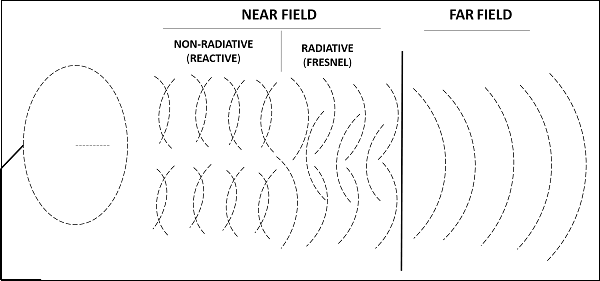The field pattern can be classified as −

• Reactive near-field region and Radiating near-field region – both termed as nearfield.
• Radiating far-field region – simply called as far-field.

The field, which is very near to the antenna is reactive near field or non-radiative field where the radiation is not pre-dominant. The region next to it can be termed as radiating near field or Fresnel’s field as the radiation predominates and the angular field distribution, depends on the physical distance from the antenna.

The region next to it is radiating far-field region. In this region, field distribution is independent of the distance from antenna. The effective radiation pattern is observed in this region.

# Antenna Theory – Radiation Pattern

Radiation is the term used to represent the emission or reception of wave front at the antenna, specifying its strength. In any illustration, the sketch drawn to represent the radiation of an antenna is its radiation pattern. One can simply understand the function and directivity of an antenna by having a look at its radiation pattern.

The power when radiated from the antenna has its effect in the near and far field regions.

• Graphically, radiation can be plotted as a function of angular position and radial distance from the antenna.
• This is a mathematical function of radiation properties of the antenna represented as a function of spherical co-ordinates, E (θ, Ø) and H (θ, Ø).

The energy radiated by an antenna is represented by the Radiation pattern of the antenna. Radiation Patterns are diagrammatical representations of the distribution of radiated energy into space, as a function of direction.

Let us look at the pattern of energy radiation.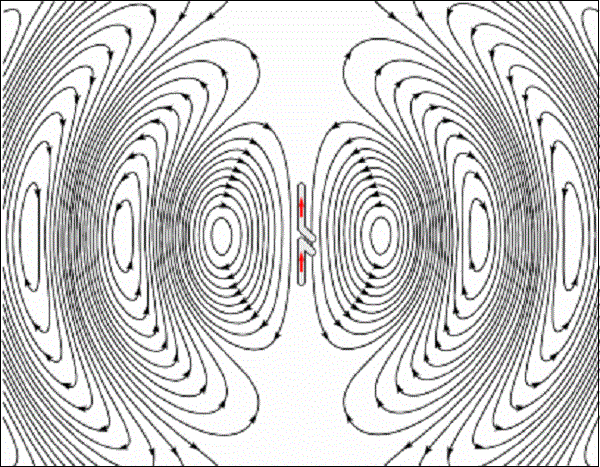The figure given above shows radiation pattern of a dipole antenna. The energy being radiated is represented by the patterns drawn in a particular direction. The arrows represent directions of radiation.

The radiation patterns can be field patterns or power patterns.

• The field patterns are plotted as a function of electric and magnetic fields. They are plotted on logarithmic scale.
• The power patterns are plotted as a function of square of the magnitude of electric and magnetic fields. They are plotted on logarithmic or commonly on dB scale.

The radiation pattern is a three-dimensional figure and represented in spherical coordinates (r, θ, Φ) assuming its origin at the center of spherical coordinate system. It looks like the following figure −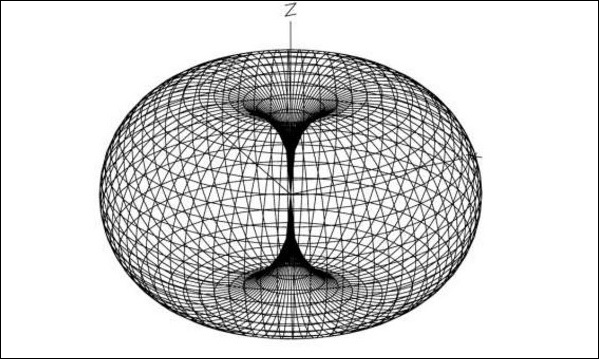The given figure is a three dimensional radiation pattern for an Omni directional pattern. This clearly indicates the three co-ordinates (x, y, z).

Two-dimensional pattern can be obtained from three-dimensional pattern by dividing it into horizontal and vertical planes. These resultant patterns are known as Horizontal pattern and Vertical pattern respectively.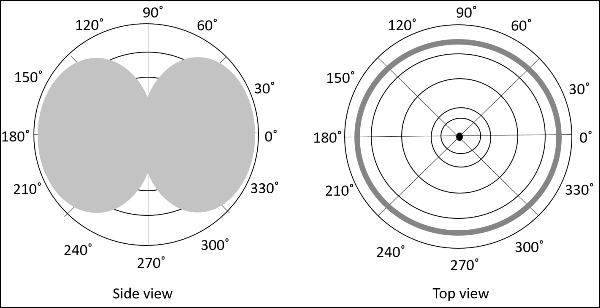The figures show the Omni directional radiation pattern in H and V planes as explained above. H-plane represents the Horizontal pattern, whereas V-plane represents the Vertical pattern.

### Lobe Formation

In the representation of radiation pattern, we often come across different shapes, which indicate the major and minor radiation areas, by which the radiation efficiency of the antenna is known.

To have a better understanding, consider the following figure, which represents the radiation pattern of a dipole antenna.Here, the radiation pattern has main lobe, side lobes and back lobe.

• The major part of the radiated field, which covers a larger area, is the main lobe or major lobe. This is the portion where maximum radiated energy exists. The direction of this lobe indicates the directivity of the antenna.
• The other parts of the pattern where the radiation is distributed side wards are known as side lobes or minor lobes. These are the areas where the power is wasted.
• There is other lobe, which is exactly opposite to the direction of main lobe. It is known as back lobe, which is also a minor lobe. A considerable amount of energy is wasted even here.

### Example

If the antennas used in radar systems produce side lobes, target tracing becomes very difficult. This is because, false targets are indicated by these side lobes. It is messy to trace out the real ones and to identify the fake ones. Hence, elimination of these side lobes is must, in order to improve the performance and save the energy.

### Remedy

The radiated energy, which is being wasted in such forms needs to be utilized. If these minor lobes are eliminated and this energy is diverted into one direction (that is towards the major lobe), then the directivity of the antenna gets increased which leads to antenna’s better performance.

The common types of Radiation patterns are −

• Omni-directional pattern (also called non-directional pattern): The pattern usually has a doughnut shape in three-dimensional view. However, in two-dimensional view, it forms a figure-of-eight pattern.
• Pencil-beam pattern − The beam has a sharp directional pencil shaped pattern.
• Fan-beam pattern − The beam has a fan-shaped pattern.
• Shaped beam pattern − The beam, which is non-uniform and patternless is known as shaped beam.

A referential point for all these types of radiation is the isotropic radiation. It is important to consider the isotropic radiation even though it is impractical.

# Antenna Theory – Isotropic Radiation

In the previous chapter, we have gone through the radiation pattern. To have a better analysis regarding the radiation of an antenna, a referential point is necessary. The radiation of an isotropic antenna, fills this space.

## Definition

Isotropic radiation is the radiation from a point source, radiating uniformly in all directions, with same intensity regardless of the direction of measurement.

The improvement of radiation pattern of an antenna is always assessed using the isotropic radiation of that antenna. If the radiation is equal in all directions, then it is known as isotropic radiation.

• The point source is an example of isotropic radiator. However, this isotropic radiation is practically impossible, because every antenna radiates its energy with some directivity.
• It has a doughnut-shaped pattern when viewed in 3D and a figure-of-eight pattern when viewed in 2D.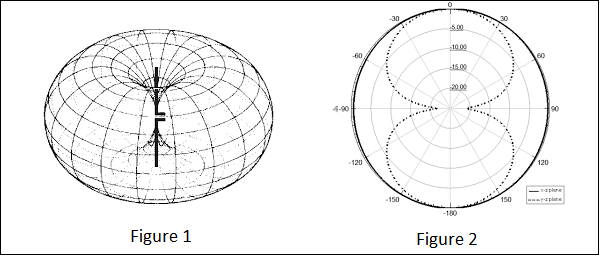The figures given above show the radiation pattern of an isotropic or Omni-directional pattern. Figure 1 illustrates the doughnut shaped pattern in 3D and Figure 2 illustrates the figure-of-eight pattern in 2D.

### Gain

The isotropic radiator has unity gain, which means having a gain factor of 1 in all directions. In terms of dB, it can be called as 0dB gain (zero loss).

According to the standard definition, “The amount of power that an isotropical antenna radiates to produce the peak power density observed in the direction of maximum antenna gain, is called as Equivalent Isotropic Radiated Power.”

If the radiated energy of an antenna is made to concentrate on one side or a particular direction, where the radiation is equivalent to that antenna’s isotropic radiated power, such a radiation would be termed as EIRP i.e. Equivalent Isotropic Radiated Power.

### Gain

Though isotropic radiation is an imaginary one, it is the best an antenna can give. The gain of such antenna will be 3dBi where 3dB is a factor of 2 and ‘i’ represents factor of isotropic condition.

If the radiation is focused in certain angle, then EIRP increases along with the antenna gain. Gain of the antenna is best achieved by focusing the antenna in certain direction.

If the radiated power is calculated by taking half-wave dipole as the reference, rather than an isotropic antenna, then it can be termed as ERP (Effective Radiated Power).

ERP(dBW)=EIRP(dBW)2.15dBiERP(dBW)=EIRP(dBW)−2.15dBi

If EIRP is known, then ERP can be calculated from formula given above.

# Antenna Theory – Beam and Polarization

This chapter deals with the parameters of radiated beam of the antenna. These parameters help us to know about the beam specifications.

## Beam Area

According to the standard definition, “Beam area is the solid angle through which all the power radiated by the antenna would stream if P (θ, Ø) maintained its maximum value over ΩA and was zero elsewhere.”

The radiated beam of the antenna comes out from an angle at the antenna, known as solid angle, where the power radiation intensity is maximum. This solid beam angle is termed as the beam area. It is represented by ΩA.

The radiation intensity P (θ, Ø) should be maintained constant and maximum throughout the solid beam angle ΩA, its value being zero elsewhere.

Beam angle is a set of angles between the half power points of the main lobe.

### Mathematical Expression

The mathematical expression for beam area is

ΩA=2π0π0Pπ(θ,Φ)dΩ watttsΩA=∫02π∫0πPπ(θ,Φ)dΩ wattts
dΩ=sinθ dθ dΦ wattsdΩ=sin⁡θ dθ dΦ watts

Where

• ΩAΩA is the solid beam angle.
• θθ is the function of angular position.
• ΦΦ is the function of radial distance.

### Units

The unit of beam area is watts.

## Beam Efficiency

According to the standard definition, “The beam efficiency states the ratio of the beam area of the main beam to the total beam area radiated.”

The energy when radiated from an antenna, is projected according to the antenna’s directivity. The direction in which an antenna radiates more power has maximum efficiency, while some of the energy is lost in side lobes. The maximum energy radiated by the beam, with minimum losses can be termed as beam efficiency.

### Mathematical Expression

The mathematical expression for beam efficiency is −

ηB=ΩMBΩAηB=ΩMBΩA

Where,

• ηBηB is the beam efficiency.
• ΩMBΩMB is beam area of the main beam.
• ΩAΩA is total solid beam angle (beam area).

## Antenna Polarization

An Antenna can be polarized depending upon our requirement. It can be linearly polarized or circularly polarized. The type of antenna polarization decides the pattern of the beam and polarization at the reception or transmission.

### Linear polarization

When a wave is transmitted or received, it may be done in different directions. The linear polarization of the antenna helps in maintaining the wave in a particular direction, avoiding all the other directions. Though this linear polarization is used, the electric field vector stays in the same plane. Hence, we use this linear polarization to improve the directivity of the antenna.

### Circular polarization

When a wave is circularly polarized, the electric field vector appears to be rotated with all its components loosing orientation. The mode of rotation may also be different at times. However, by using circular polarization, the effect of multi-path gets reduced and hence it is used in satellite communications such as GPS.

### Horizontal polarization

Horizontal polarization makes the wave weak, as the reflections from the earth surface affect it. They are usually weak at low frequencies below 1GHz. Horizontal polarization is used in the transmission of TV signals to achieve a better signal to noise ratio.

### Vertical polarization

The low frequency vertically polarized waves are advantageous for ground wave transmission. These are not affected by the surface reflections like the horizontally polarized ones. Hence, the vertical polarization is used for mobile communications.

Each type of polarization has its own advantages and disadvantages. A RF system designer is free to select the type of polarization, according to the system requirements.

# Antenna Theory – Beam Width

In this chapter, we shall discuss about another important factor in the radiation pattern of an antenna, known as beam width. In the radiation pattern of an antenna, the main lobe is the main beam of the antenna where maximum and constant energy radiated by the antenna flows.

Beam width is the aperture angle from where most of the power is radiated. The two main considerations of this beam width are Half Power Beam Width (HPBW) and First Null Beam Width (FNBW).

## Half-Power Beam Width

According to the standard definition, “The angular separation, in which the magnitude of the radiation pattern decreases by 50% (or -3dB) from the peak of the main beam, is the Half Power Beam Width.”

In other words, Beam width is the area where most of the power is radiated, which is the peak power. Half power beam width is the angle in which relative power is more than 50% of the peak power, in the effective radiated field of the antenna.

### Indication of HPBW

When a line is drawn between radiation pattern’s origin and the half power points on the major lobe, on both the sides, the angle between those two vectors is termed as HPBW, half power beam width. This can be well understood with the help of the following diagram.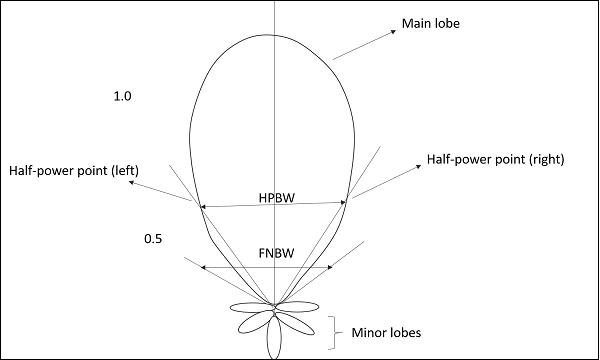The figure shows half-power points on the major lobe and HPBW.

### Mathematical Expression

The mathematical expression for half power beam width is −

HalfpowerBeamwith=70λ/DHalfpowerBeamwith=70λ/D

Where

• λλ is wavelength (λ = 0.3/frequency).
• D is Diameter.

### Units

The unit of HPBW is radians or degrees.

## First Null Beam Width

According to the standard definition, “The angular span between the first pattern nulls adjacent to the main lobe, is called as the First Null Beam Width.”

Simply, FNBW is the angular separation, quoted away from the main beam, which is drawn between the null points of radiation pattern, on its major lobe.

### Indication of FNBW

Draw tangents on both sides starting from the origin of the radiation pattern, tangential to the main beam. The angle between those two tangents is known as First Null Beam Width (FNBW).

This can be better understood with the help of the following diagram.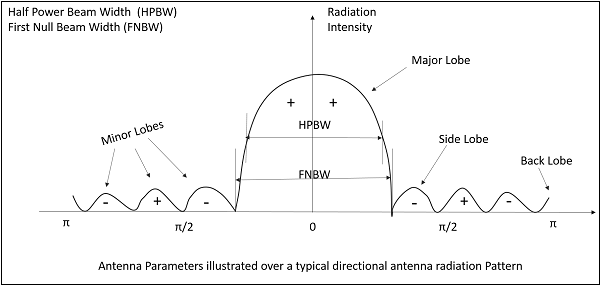The above image shows the half power beam width and first null beam width, marked in a radiation pattern along with minor and major lobes.

### Mathematical Expression

The mathematical expression of First Null Beam Width is

FNBW=2HPBWFNBW=2HPBW
FNBW2(70λ/D)=140λ/DFNBW2(70λ/D)=140λ/D

Where

• λλ is wavelength (λ = 0.3/frequency).
• D is Diameter.

### Units

The unit of FNBW is radians or degrees.

## Effective Length & Effective Area

Among the antenna parameters, the effective length and effective area are also important. These parameters help us to know about the antenna’s performance.

### Effective length

Antenna Effective length is used to determine the polarization efficiency of the antenna.

Definition− “The Effective length is the ratio of the magnitude of voltage at the open terminals of the receiving antenna to the magnitude of the field strength of the incident wave front, in the same direction of antenna polarization.”

When an incident wave arrives at the antenna’s input terminals, this wave has some field strength, whose magnitude depends upon the antenna’s polarization. This polarization should match with the magnitude of the voltage at receiver terminals.

### Mathematical Expression

The mathematical expression for effective length is −

le=VocEile=VocEi

Where

• lele is the effective length.
• VocVoc is open-circuit voltage.
• EiEi is the field strength of the incident wave.

### Effective area

Definition − “Effective area is the area of the receiving antenna, which absorbs most of the power from the incoming wave front, to the total area of the antenna, which is exposed to the wave front.”

The whole area of an antenna while receiving, confronts the incoming electromagnetic waves, whereas only some portion of the antenna, receives the signal, known as the effective area.

Only some portion of the received wave front is utilized because some portion of the wave gets scattered while some gets dissipated as heat. Hence, without considering the losses, the area, which utilizes the maximum power obtained to the actual area, can be termed as effective area.

Effective area is represented by AeffAeff.

# Antenna Theory – Reciprocity

An antenna can be used as both transmitting antenna and receiving antenna. While using so, we may come across a question whether the properties of the antenna might change as its operating mode is changed. Fortunately, we need not worry about that. The properties of antenna being unchangeable is called as the property of reciprocity.

## Properties under Reciprocity

The properties of transmitting and receiving antenna that exhibit the reciprocity are −

• Equality of Directional patterns.
• Equality of Directivities.
• Equality of Effective lengths.
• Equality of Antenna impedances.

Let us see how these are implemented.

### Equality of Directional patterns

The radiation pattern of transmitting antenna1, which transmits to the receiving antenna2 is equal to the radiation pattern of antenna2, if it transmits and antenna1 receives the signal.

### Equality of Directivities

Directivity is same for both transmitting and receiving antennas, if the value of directivity is same for both the cases i.e. the directivities are same whether calculated from transmitting antenna’s power or receiving antenna’s power.

### Equality of Effective lengths

The value of maximum effective aperture is same for both transmitting and receiving antennas. Equality in the lengths of both transmitting and receiving antennas is maintained according to the value of the wavelength.

### Equality in Antenna Impedances

The output impedance of a transmitting antenna and the input impedance of a receiving antenna are equal in an effective communication.

These properties will not change though the same antenna is operated as a transmitter or as a receiver. Hence, the property of reciprocity is followed.

# Antenna Theory – Poynting Vector

Antennas radiate Electromagnetic energy to transmit or to receive information. Therefore, the terms Energy and Power are associated with these electromagnetic waves and we have to discuss them. An electromagnetic wave has both electric and magnetic fields.

Consider the wave at any instant, which can be viewed in both the vectors. The following figure shows the representation of electric and magnetic field components in an Electromagnetic wave.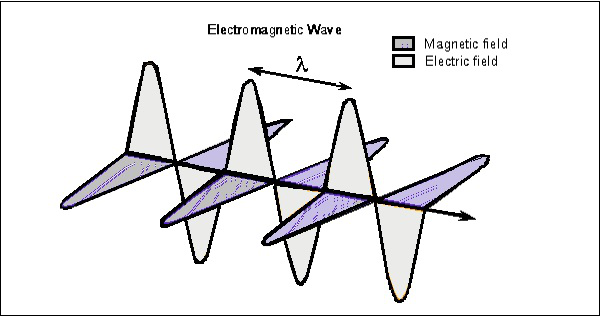The electric wave is present vertical to the propagation of EM wave, while the magnetic wave is horizontally located. Both the fields are at right angles to each other.

## Poynting Vector

Poynting vector describes the energy of the EM Wave per unit time per unit area at any given instant of time. John Henry Poynting first derived this vector in 1884 and hence it was named after him.

Definition − “Poynting vector gives the rate of energy transfer per unit area”

or

“The energy that a wave carries per unit time per unit area is given by the Poynting vector.”

Poynting vector is represented by Ŝ.

### Units

The SI unit of Poynting vector is W/m2.

### Mathematical Expression

The quantity that is used to describe the power associated with the electromagnetic waves is the instantaneous Poynting vector, which is defined as

S^=E^×H^S^=E^×H^

Where

• S^S^ is the instantaneous Poynting vector (W/m2).
• E^E^ is the instantaneous electric field intensity (V/m).
• H^H^ is the instantaneous magnetic field intensity (A/m).

The important point to be noted here is that the magnitude of E is greater than H within an EM wave. However, both of them contribute the same amount of energy. Ŝ is the vector, which has both direction and magnitude. The direction of Ŝ is same as the velocity of the wave. Its magnitude depends upon the E and H.

## Derivation of Poynting Vector

To have a clear idea on Poynting vector, let us go through the derivation of this Poynting vector, in a step-by-step process.

Let us imagine that an EM Wave, passes an area (A) perpendicular to the X-axis along which the wave travels. While passing through A, in infinitesimal time (dt), the wave travels a distance (dx).

dx=C dtdx=C dt

Where

C=velocity of light=3×108m/sC=velocity of light=3×108m/s
dμ=μ dv=(ϵ0E2)(AC dt)dμ=μ dv=(ϵ0E2)(AC dt)
=ϵ0AC E2 dt=ϵ0AC E2 dt

Therefore, Energy transferred in time (dt) per area (A) is −

S=EnergyTime×Area=dWdt A=ϵ0ACE2 dtdt A=ϵ0CE2S=EnergyTime×Area=dWdt A=ϵ0ACE2 dtdt A=ϵ0CE2

Since

EH=μ0ϵ0−−−√ then S=CB2μ0EH=μ0ϵ0 then S=CB2μ0

Since

C=EH then S=EBμ0C=EH then S=EBμ0
=S^=1μ0(E^H^)=S^=1μ0(E^H^)

Ŝ denotes the Poynting vector.

The above equation gives us the energy per unit time, per unit area at any given instant of time, which is called as Poynting vector.

# Antenna Theory – Types of Antennas

Antennas have to be classified to understand their physical structure and functionality more clearly. There are many types of antennas depending upon the applications applications.

Type of antenna Examples Applications
Wire Antennas Dipole antenna, Monopole antenna, Helix antenna, Loop antenna Personal applications, buildings, ships, automobiles, space crafts
Aperture Antennas Waveguide (opening), Horn antenna Flush-mounted applications, air-craft, space craft
Reflector Antennas Parabolic reflectors, Corner reflectors Microwave communication, satellite tracking, radio astronomy
Lens Antennas Convex-plane, Concave-plane, Convex-convex, Concaveconcave lenses Used for very highfrequency applications
Micro strip Antennas Circular-shaped, Rectangularshaped metallic patch above the ground plane Air-craft, space-craft, satellites, missiles, cars, mobile phones etc.
Array Antennas Yagi-Uda antenna, Micro strip patch array, Aperture array, Slotted wave guide array Used for very high gain applications, mostly when needs to control the radiation pattern

Let us discuss the above-mentioned types of antennas in detail, in the coming chapters.

# Antenna Theory – Wire

Wire antennas are the basic types of antennas. These are well known and widely used antennas. To have a better idea of these wire antennas, first let us have a look at the transmission lines.

## Transmission Lines

The wire or the transmission line has some power, which travels from one end to the other end. If both the ends of transmission line are connected to circuits, then the information will be transmitted or received using this wire between these two circuits.

If one end of this wire is not connected, then the power in it tries to escape. This leads to wireless communication. If one end of the wire is bent, then the energy tries to escape from the transmission line, more effectively than before. This purposeful escape is known as Radiation.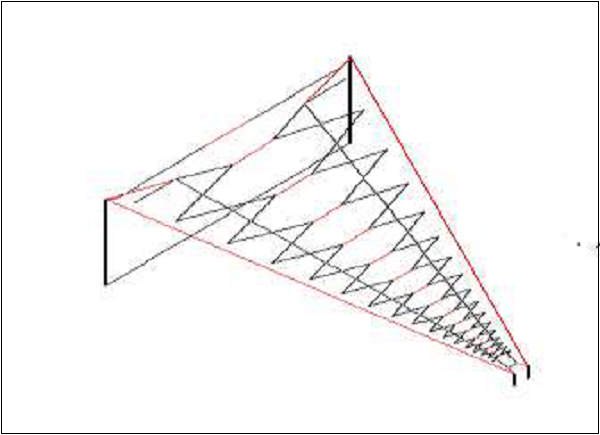For the radiation to take place effectively, the impedance of the open end of the transmission line should match with the impedance of the free-space. Consider a transmission line of a quarter-wave length size. The far end of it is kept open and bent to provide high impedance. This acts as a half-wave dipole antenna. Already, it has low impedance at one end of the transmission line. The open end, which has high impedance, matches with the impedance of free space to provide better radiation.

### Dipole

The radiation of energy when done through such a bent wire, the end of such transmission line is termed as dipole or dipole antenna.

The reactance of the input impedance is a function of the radius and length of the dipole. The smaller the radius, the larger the amplitude of the reactance. It is proportional to the wavelength. Hence, the length and radius of the dipole should also be taken into consideration. Normally, its impedance is around 72Ω.

This is better understood with the help of the following figure.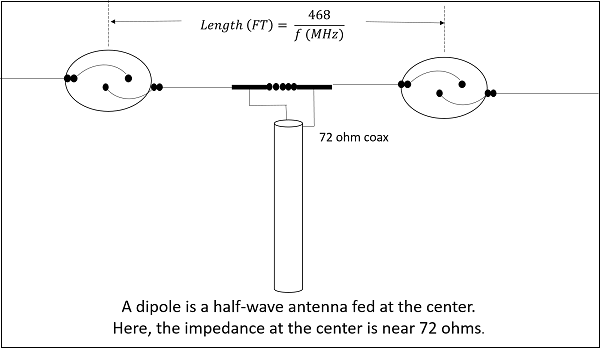The figure shows the circuit diagram of a normal dipole connected to a transmission line. The current for a dipole is maximum at the center and minimum at its ends. The voltage is minimum at its center and maximum at its ends.

The types of wire antennas include Half-wave dipole, Half-wave folded dipole, Full-wave dipole, Short dipole, and Infinitesimal dipole. All of these antennas will be discussed in further chapters.

# Antenna Theory – Half-Wave Dipole

The dipole antenna is cut and bent for effective radiation. The length of the total wire, which is being used as a dipole, equals half of the wavelength (i.e., l = λ/2). Such an antenna is called as half-wave dipole antenna. This is the most widely used antenna because of its advantages. It is also known as Hertz antenna.

### Frequency range

The range of frequency in which half-wave dipole operates is around 3KHz to 300GHz. This is mostly used in radio receivers.

## Construction & Working of Half-wave Dipole

It is a normal dipole antenna, where the frequency of its operation is half of its wavelength. Hence, it is called as half-wave dipole antenna.

The edge of the dipole has maximum voltage. This voltage is alternating (AC) in nature. At the positive peak of the voltage, the electrons tend to move in one direction and at the negative peak, the electrons move in the other direction. This can be explained by the figures given below.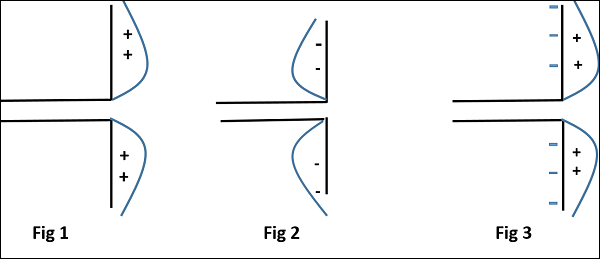The figures given above show the working of a half-wave dipole.

• Fig 1 shows the dipole when the charges induced are in positive half cycle. Now the electrons tend to move towards the charge.
• Fig 2 shows the dipole with negative charges induced. The electrons here tend to move away from the dipole.
• Fig 3 shows the dipole with next positive half cycle. Hence, the electrons again move towards the charge.

The cumulative effect of this produces a varying field effect which gets radiated in the same pattern produced on it. Hence, the output would be an effective radiation following the cycles of the output voltage pattern. Thus, a half-wave dipole radiates effectively.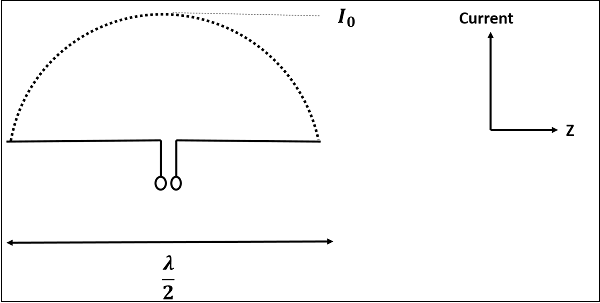The above figure shows the current distribution in half wave dipole. The directivity of half wave dipole is 2.15dBi, which is reasonably good. Where, ‘i’ represents the isotropic radiation.

The radiation pattern of this half-wave dipole is Omni-directional in the H-plane. It is desirable for many applications such as mobile communications, radio receivers etc.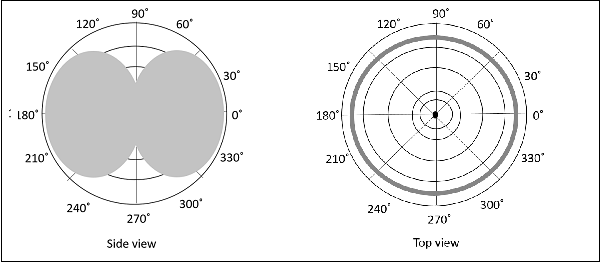The above figure indicates the radiation pattern of a half wave dipole in both H-plane and V-plane.

The radius of the dipole does not affect its input impedance in this half wave dipole, because the length of this dipole is half wave and it is the first resonant length. An antenna works effectively at its resonant frequency, which occurs at its resonant length.

The following are the advantages of half-wave dipole antenna −

• Input impedance is not sensitive.
• Matches well with transmission line impedance.
• Has reasonable length.
• Length of the antenna matches with size and directivity.

The following are the disadvantages of half-wave dipole antenna −

• Not much effective due to single element.
• It can work better only with a combination.

### Applications

The following are the applications of half-wave dipole antenna −

• When employed with others, used for wide variety of applications.

# Antenna Theory – Half-Wave Folded Dipole

A folded dipole is an antenna, with two conductors connected on both sides, and folded to form a cylindrical closed shape, to which feed is given at the center. The length of the dipole is half of the wavelength. Hence, it is called as half wave folded dipole antenna.

### Frequency range

The range of frequency in which half wave folded dipole operates is around 3KHz to 300GHz. This is mostly used in television receivers.

## Construction & Working of Half-wave Folded Dipole

This antenna is commonly used with the array type antennas to increase the feed resistance. The most commonly used one is with Yagi-Uda antenna. The following figure shows a half-wave folded dipole antenna.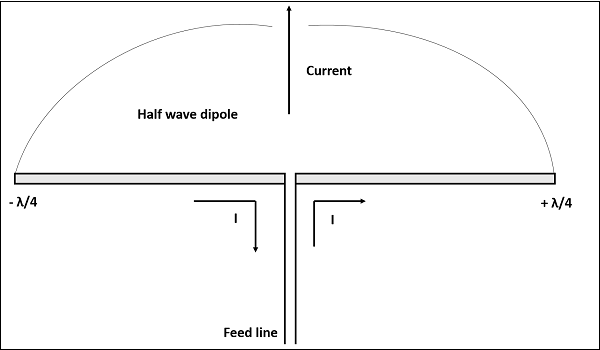This antenna uses an extra conducting element (a wire or a rod) when compared with previous dipole antenna. This is continued by placing few conducting elements in parallel, with insulation in-between, in array type of antennas.

The following figure explains the working of a half-wave folded dipole antenna, when it is provided with excitation.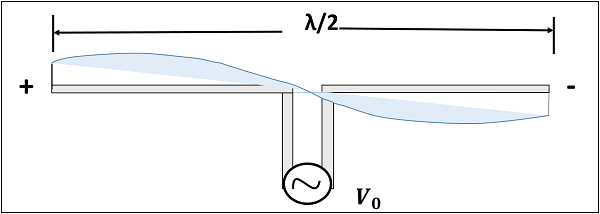If the diameter of the main conductor and the folded dipole are same, then there will be four folded (two times of squared one) increase in the feed impedance of the antenna. This increase in feed impedance is the main reason for the popular usage of this folded dipole antenna. Due of the twin-lead, the impedance will be around 300Ω.

The radiation pattern of half-wave folded dipoles is the same as that of the half-wave dipole antennas. The following figure shows the radiation pattern of half-wave folded dipole antenna, which is Omni-directional pattern.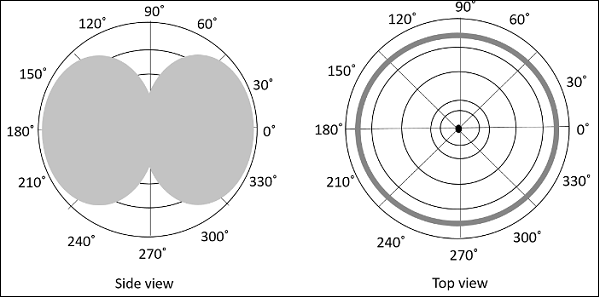Half-wave folded dipole antennas are used where optimum power transfer is needed and where large impedances are needed.

This folded dipole is the main element in Yagi-Uda antenna. The following figure shows a Yagi-Uda antenna, which we will study later. The main element used here is this folded dipole, to which the antenna feed is given. This antenna has been used extensively for television reception over the last few decades.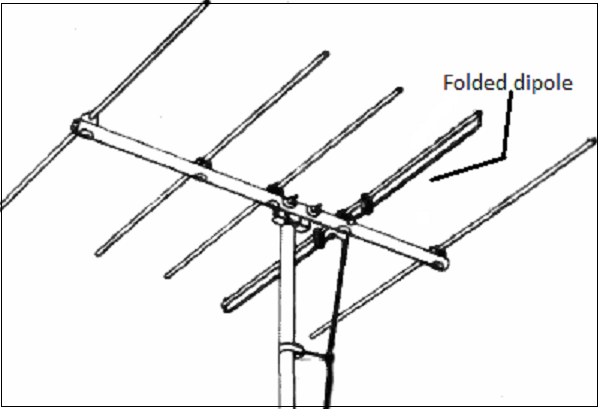The following are the advantages of half-wave folded dipole antenna −

• Reception of balanced signals.
• Receives a particular signal from a band of frequencies without losing the quality.
• A folded dipole maximizes the signal strength.

The following are the disadvantages of half-wave folded dipole antenna −

• Displacement and adjustment of antenna is a hassle.
• Outdoor management can be difficult when antenna size increases.

### Applications

The following are the applications of half-wave folded dipole antenna −

• Mainly used as a feeder element in Yagi antenna, Parabolic antenna, turnstile antenna, log periodic antenna, phased and reflector arrays, etc.
• Most commonly used in TV receiver antennas.

# Antenna Theory – Full-Wave Dipole

If the length of the dipole, i.e. the total wire, equals the full wavelength λ, then it is called as full wave dipole. If a full wavelength dipole is used either for transmission or for reception, let us see how the radiation will be.

## Construction & Working of Full-wave Dipole

The full-wave dipole with its voltage and current distribution is shown here. Both the positive and negative peaks of the wave induce positive and negative voltages respectively. However, as the induced voltages cancel out each other, there is no question of radiation.

The above figure shows the voltage distribution of full-wave dipole whose length is λ. It is seen that two half-wave dipoles are joined to make a full-wave dipole.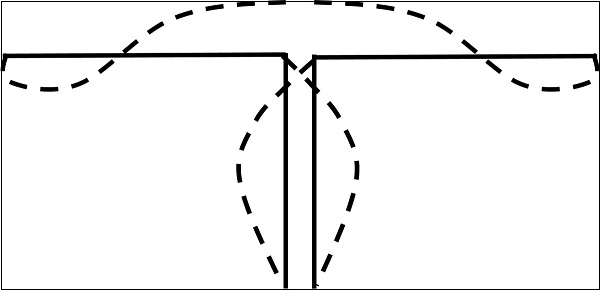The voltage pattern when induces its positive charges and negative charges at the same time, cancel out each other as shown in the figure. The induced charges make no further attempt of radiation since they are cancelled. The output radiation will be zero for a fullwave transmission dipole.

As there is no radiation pattern, no directivity and no gain, the Full wave dipole is seldom used as an antenna. Which means, though the antenna radiates, it is just some heat dissipation, which is a wastage of power.

The following are the disadvantages of full-wave dipole antenna.

• Heat dissipation
• Wastage of power
• No directivity and no gain

Due to these drawbacks, the full-wave dipole is seldom used.

# Antenna Theory – Short Dipole

short dipole is a simple wire antenna. One end of it is open-circuited and the other end is fed with AC source. This dipole got its name because of its length.

### Frequency range

The range of frequency in which short dipole operates is around 3KHz to 30MHz. This is mostly used in low frequency receivers.

## Construction & Working of Short Dipole

The Short dipole is the dipole antenna having the length of its wire shorter than the wavelength. A voltage source is connected at one end while a dipole shape is made, i.e., the lines are terminated at the other end.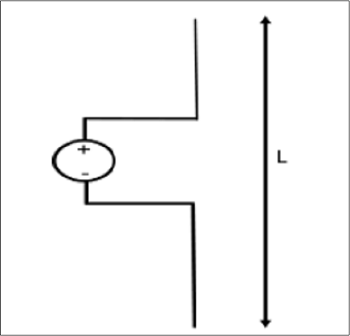The circuit diagram of a short dipole with length L is shown. The actual size of the antenna does not matter. The wire that leads to the antenna must be less than one-tenth of the wavelength. That is

L<λ10L<λ10

Where

• L is the length of the wire of the short dipole.
• λ is the wavelength.

Another type of short dipole is infinitesimal dipole, whose length is far less than its wave length. Its constructiion is similar to it, but uses a capacitor plate.

## Infinitesimal Dipole

A dipole whose length is far less than wavelength is infitesimal dipole. This antenna is actually impractical. Here, the length of the dipole is less than even fiftith part of the wavelength.

The length of the dipole, Δl << λ. Where, λ is the wavelength.

Δl=λ50Δl=λ50

Hence, this is the infinitely small dipole, as the name implies.

As the length of these dipoles is very small, the current flow in the wire will be dI. These wires are generally used with capacitor plates on both sides, where low mutual coupling is needed. Because of the capacitor plates, we can say that uniform distribution of current is present. Hence the current is not zero here.

The capacitor plates can be simply conductors or the wire equivalents. The fields radiated by the radial currents tend to cancel each other in the far field so that the far fields of the capacitor plate antenna can be approximated by the infinitesimal dipole.

The radiation pattern of a short dipole and infinitesimal dipole is similar to a half wave dipole. If the dipole is vertical, the pattern will be circular. The radiation pattern is in the shape of “figure of eight” pattern, when viewed in two-dimensional pattern.

The following figure shows the radiation pattern of a short dipole antenna, which is in omni-directional pattern.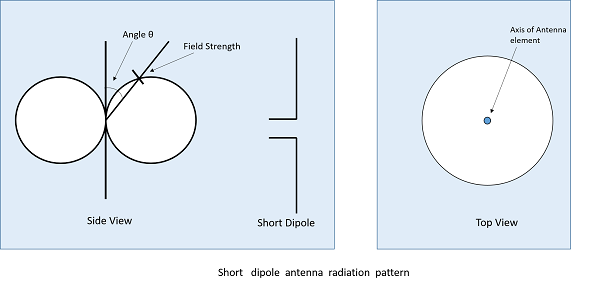The following are the advantages of short dipole antenna −

• Ease of construction, due to small size
• Power dissipation efficiency is higher

The following are the disadvantages of short dipole antenna −

• High resistive losses
• High power dissipation
• Low Signal-to-noise ratio
• Not so efficient

### Applications

The following are the applications of short dipole antenna −

• Used in narrow band applications.
• Used as an antenna for tuner circuits.

In this chapter, the popular and most widely used short-wire antennas were discussed. We will discuss the Long-wire antennas in the coming chapters.

# Antenna Theory – Long-Wire

We have gone through different types of short wire antennas. Now, lets us look at the long wire antennas. The long wire antennas are formed by using a number of dipoles. The length of the wire in these type of antennas is n times λ/2

L=n λ/2L=n λ/2

Where,

• L is the length of the antenna,
• n is the number of elements,
• λ is the wavelength

As ‘n’ increases, the directional properties also increase.

## Types of Long-wire Antennas

Long wire antennas are divided into two types namely − Resonant Antennas and Non-resonant Antennas.

### Resonant Antennas

Resonant Antennas are those for which a sharp peak in the radiated power is intercepted by the antenna at certain frequency, to form a standing wave. The radiation pattern of the radiated wave is not matched with the load impedance in this type of antenna.

The resonant antennas are periodic in nature. They are also called as bi-directional travelling wave antennas, as the radiated wave moves in two directions, which means both incident and reflected waves occur here. In these antennas, the length of the antenna and frequency are proportional to each other.

### Non-resonant Antennas

Non-resonant Antennas are those for which resonant frequency does not occur. The wave moves in forward direction and hence do not form a standing wave. The radiation pattern of the radiated wave matches with the load impedance in the non-resonant antennas.

These non-resonant antennas are non-periodic in nature. They are also called as Unidirectional travelling wave antennas, as the radiated wave moves in forward direction only, which means that only incident wave is present. As the frequency increases, the length of the antenna decreases and vice versa. Hence, the frequency and length are inversely proportional to each other.

These long-wire antennas are the basic elements for the construction of V-shaped antennas or the Rhombic antennas.

# Antenna Theory – V-Antennas

A better version of long-wire antennas is the V-Antenna. This antenna is formed by arranging the long wire in a V-shaped pattern. The end wires are called as legs. This antenna is a bi-directional resonant antenna.

### Frequency Range

The frequency range of operation of V-antenna is around 3 to 30 MHz. This antenna works in high frequency range.

## Construction & Working of V-Antennas

Two long wires are connected in the shape of V to make a V-antenna. The two long wires are excited with 180˚ out of phase. As the length of these wires increases, the gain and directivity also increases.

The following figure shows a V-antenna with the transmission line impedance z and the lengh of the wire λ/2, making an angle Φm with the axis, which is called as apex angle.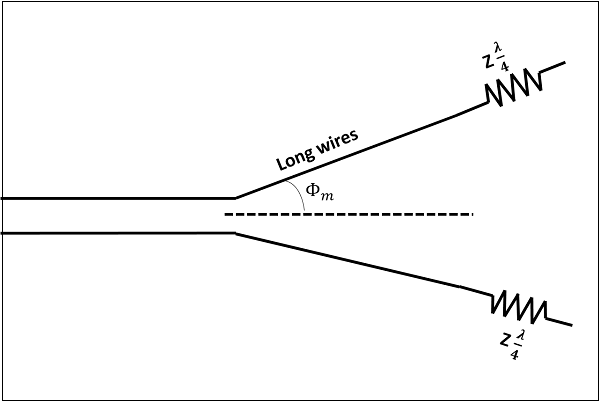The gain achieved by V-antenna is higher than normal single long wire antenna. The gain in this V-formation is nearly twice compared to the single long wire antenna, which has a length equal to the legs of V-antenna. If wide range of radiation is to be achieved, the apex angle should have an average value between higher and lower frequencies in terms of the number of λ/2 in each leg.

The radiation pattern of a V-antenna is bi-directional. The radiation obtained on each transmission line is added to obtain the resultant radiation pattern. This is well explained in the following figure −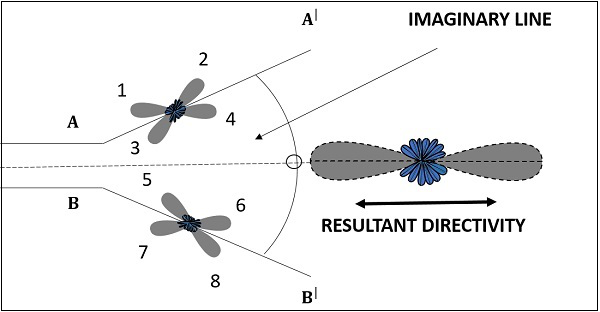The figure shows the radiation pattern of V-antenna. The two transmission lines forming V-pattern are AA’ and BB’. The patterns of individual transmission lines and the resultant pattern are shown in the figure. The resultant pattern is shown along the axis. This pattern resembles the broad-side array.

If another V-antenna is added to this antenna and fed with 90˚ phase difference, then the resultant pattern would be end-fire, doubling the power gain. The directivity is further increased by adding the array of V-antennas.

The following are the advantages of V-antenna −

• Construction is simple
• High gain
• Low manufacturing cost

The following are the disadvantages of V-antenna −

• Standing waves are formed
• The minor lobes occurred are also strong
• Used only for fixed frequency operations

### Applications

The following are the applications of V-antenna −

• Used for commercial purposes

# Antenna Theory – Inverted V-Antenna

In the previous chapter, we have studied V-antenna. Its operating frequency is limited. This can be modified by using another antenna, which is a non-resonant antenna or a travelling wave antenna. A travelling wave antenna produces no standing wave, as discussed previously.

### Frequency Range

The frequency range of operation of an inverted vee antenna (or V-antenna) is around 3 to 30 MHz. This antenna works in high frequency range.

## Construction & Working of Inverted V-Antenna

A travelling wave antenna, used in high-frequency band is an inverted V-antenna. This inverted V-antenna is easily installed on a non-conducting mast.

Take a look at the following image. It shows an inverted V-antenna mounted on a roof top.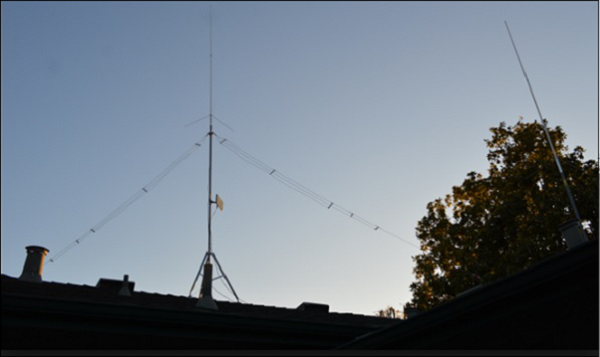The maximum radiation for an inverted V-antenna is at its center. It is similar to a halfwave dipole antenna. The antenna is placed in the shape of an inverted V, with its two transmission lines or legs bent towards the ground making 120° or 90° angle between them. The center of the antenna should not be higher than λ/4.

The angle made by one of the legs with the axis of the antenna, is known as the tilt angle and is denoted by θ.

The radiation pattern of inverted V-antenna is uni-directional pattern, as no standing waves are formed here. It can be clearly understood by the radiation pattern shown below.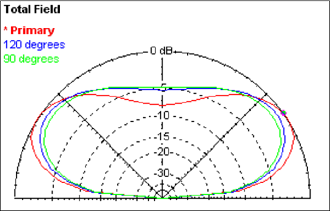The figure illustrates the radiation pattern of an inverted V-antenna. Primary radiated field is shown along with the fields when the tilt angles are 120˚ and 90˚ in the figure given above. The gain and directivity are improved by having an array of antennas.

The following are the advantages of inverted V-antenna −

• Occupies less horizontal place
• No standing waves are formed
• High gain

The following are the disadvantages of inverted V-antenna −

• It has considerable undesired minor lobes
• Minor lobes create horizontally polarized waves

### Applications

The following are the applications of inverted V-antenna −

• Used in tuned circuit applications
• Used in commercial applications

After the V-antenna and inverted V-antenna, another important long wire antenna is the Rhombic antenna. It is a combination of two V-antennas. This is discussed in the next chapter.

# Antenna Theory – Rhombic

The Rhombic Antenna is an equilateral parallelogram shaped antenna. Generally, it has two opposite acute angles. The tilt angle, θ is approximately equal to 90° minus the angle of major lobe. Rhombic antenna works under the principle of travelling wave radiator. It is arranged in the form of a rhombus or diamond shape and suspended horizontally above the surface of the earth.

### Frequency Range

The frequency range of operation of a Rhombic antenna is around 3MHz to 300MHz. This antenna works in HF and VHF ranges.

## Construction of Rhombic Antenna

Rhombic antenna can be regarded as two V-shaped antennas connected end-to-end to form obtuse angles. Due to its simplicity and ease of construction, it has many uses −

• In HF transmission and reception
• Commercial point-to-point communication

The construction of the rhombic antenna is in the form a rhombus, as shown in the figure.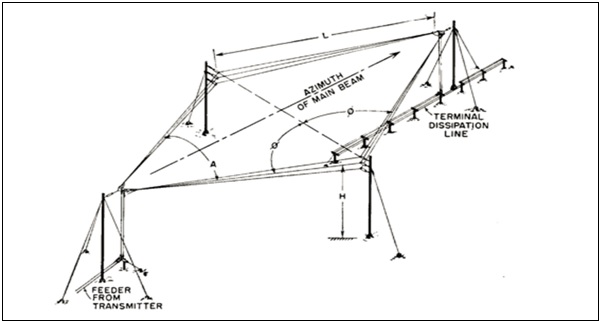The two sides of rhombus are considered as the conductors of a two-wire transmission line. When this system is properly designed, there is a concentration of radiation along the main axis of radiation. In practice, half of the power is dissipated in the terminating resistance of the antenna. The rest of the power is radiated. The wasted power contributes to the minor lobes.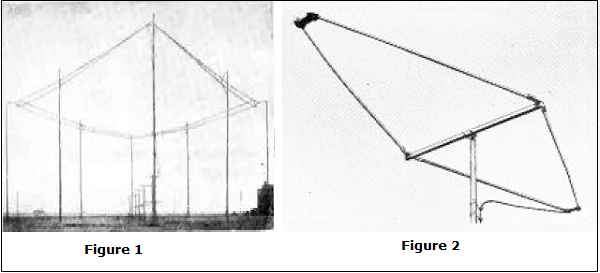Figure 1 shows the construction of rhombic antenna for point-to-point communication in olden days. Figure 2 shows the rhombic UHF antenna for TV reception, used these days.

The maximum gain from a rhombic antenna is along the direction of the main axis, which passes through the feed point to terminate in free space. The polarization obtained from a horizontal rhombic antenna is in the plane of rhombus, which is horizontal.

The radiation pattern of the rhombic antenna is shown in the following figure. The resultant pattern is the cumulative effect of the radiation at all four legs of the antenna. This pattern is uni-directional, while it can be made bi-directional by removing the terminating resistance.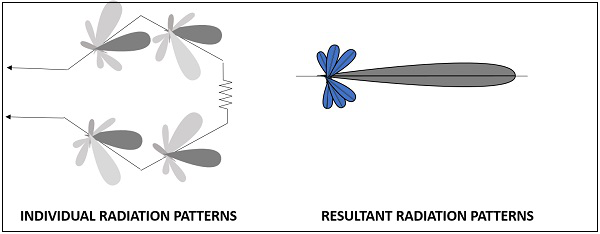The main disadvantage of rhombic antenna is that the portions of the radiation, which do not combine with the main lobe, result in considerable side lobes having both horizontal and vertical polarization.

The following are the advantages of Rhombic antenna −

• Input impedance and radiation pattern are relatively constant
• Multiple rhombic antennas can be connected
• Simple and effective transmission

The following are the disadvantages of Rhombic antenna −

• Wastage of power in terminating resistor
• Requirement of large space
• Redued transmission efficiency

### Applications

The following are the applications of Rhombic antenna −

• Used in HF communications
• Used in Long distance sky wave propagations
• Used in point-to-point communications

Another method of using long wire is by bending and making the wire into a loop shaped pattern and observing its radiational parameters. This type of antennas are termed as loop antennas.

# Antenna Theory – Loop

An RF current carrying coil is given a single turn into a loop, can be used as an antenna called as loop antenna. The currents through this loop antenna will be in phase. The magnetic field will be perpendicular to the whole loop carrying the current.

### Frequency Range

The frequency range of operation of loop antenna is around 300MHz to 3GHz. This antenna works in UHF range.

## Construction & Working of Loop Antennas

A loop antenna is a coil carrying radio frequency current. It may be in any shape such as circular, rectangular, triangular, square or hexagonal according to the designer’s convenience.

Loop antennas are of two types.

• Large loop antennas
• Small loop antennas

### Large loop antennas

Large loop antennas are also called as resonant antennas. They have high radiation efficiency. These antennas have length nearly equal to the intended wavelength.

L=λL=λ

Where,

• L is the length of the antenna
• λ is the wavelength

The main parameter of this antenna is its perimeter length, which is about a wavelength and should be an enclosed loop. It is not a good idea to meander the loop so as to reduce the size, as that increases capacitive effects and results in low efficiency.

### Small loop antennas

Small loop antennas are also called as magnetic loop antennas. These are less resonant. These are mostly used as receivers.

These antennas are of the size of one-tenth of the wavelength.

L=λ10L=λ10

Where,

• L is the length of the antenna
• λ is the wavelength

The features of small loop antennas are −

• A small loop antenna has low radiation resistance. If multi-turn ferrite core constructions are used, then high radiation resistance can be achieved.
• It has low radiation efficiency due to high losses.
• Its construction is simple with small size and weight.

Due to its high reactance, its impedance is difficult to match with the transmitter. If loop antenna have to act as transmitting antenna, then this impedance mis-match would definitely be a problem. Hence, these loop antennas are better operated as receiver antennas.

## Frequently Used Loops

Small loop antennas are mainly of two types −

• Circular loop antennas
• Square loop antennas

These two types of loop antennas are mostly widely used. Other types (rectangular, delta, elliptical etc.) are also made according to the designer specifications.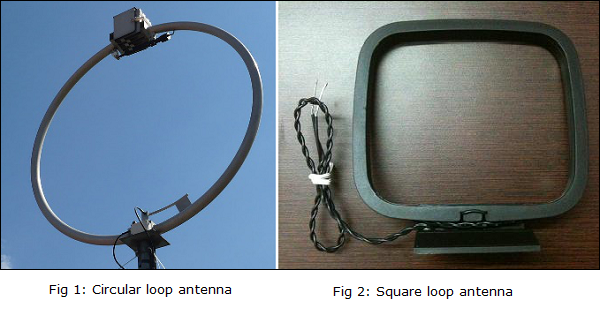The above images show circular and square loop antennas. These types of antennas are mostly used as AM receivers because of high Signal-to-noise ratio. They are also easily tunable at the Q-tank circuit in radio receivers.

## Polarization of Loop

The polarization of the loop antenna will be vertically or horizontally polarized depending upon the feed position. The vertical polarization is given at the center of the vertical side while the horizontal polarization is given at the center of the horizontal side, depending upon the shape of the loop antenna.

The small loop antenna is generally a linearly polarized one. When such a small loop antenna is mounted on top of a portable receiver, whose output is connected to a meter, it becomes a great direction finder.

The radiation pattern of these antennas will be same as that of short horizontal dipole antenna.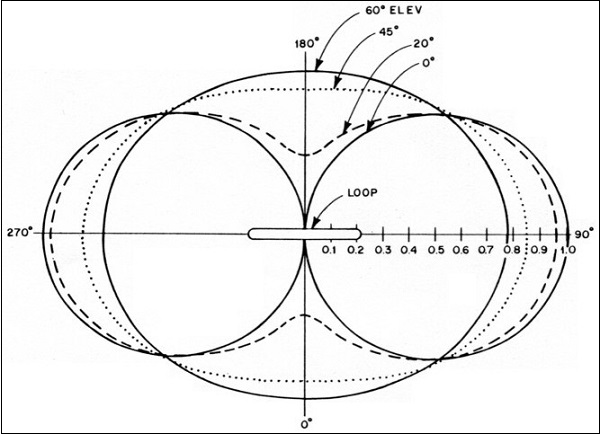The radiation pattern for small, high-efficiency loop antennas is shown in the figure given above. The radiation patterns for different angles of looping are also illustrated clearly in the figure. The tangent line at 0° indicates vertical polarization, whereas the line with 90° indicates horizontal polarization.

The following are the advantages of Loop antenna −

• Compact in size
• High directivity

The following are the disadvantages of Loop antenna −

• Impedance matching may not be always good
• Has very high resonance quality factor

### Applications

The following are the applications of Loop antenna −

• Used in RFID devices
• Used in MF, HF and Short wave receivers
• Used in Aircraft receivers for direction finding
• Used in UHF transmitters

# Antenna Theory – Helical

Helical antenna is an example of wire antenna and itself forms the shape of a helix. This is a broadband VHF and UHF antenna.

### Frequency Range

The frequency range of operation of helical antenna is around 30MHz to 3GHz. This antenna works in VHF and UHF ranges.

## Construction & Working of Helical Antenna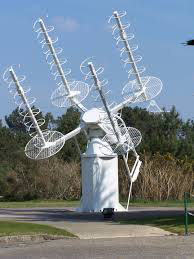Helical antenna or helix antenna is the antenna in which the conducting wire is wound in helical shape and connected to the ground plate with a feeder line. It is the simplest antenna, which provides circularly polarized waves. It is used in extra-terrestrial communications in which satellite relays etc., are involved.

The above image shows a helical antenna system, which is used for satellite communications. These antennas require wider outdoor space.

It consists of a helix of thick copper wire or tubing wound in the shape of a screw thread used as an antenna in conjunction with a flat metal plate called a ground plate. One end of the helix is connected to the center conductor of the cable and the outer conductor is connected to the ground plate.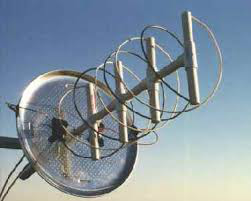The image of a helix antenna detailing the antenna parts is shown above.

The radiation of helical antenna depends on the diameter of helix, the turn spacing and the pitch angle.

Pitch angle is the angle between a line tangent to the helix wire and plane normal to the helix axis.

α=tan1(SπD)α=tan−1⁡(SπD)

where,

• D is the diameter of helix.
• S is the turn spacing (centre to centre).
• α is the pitch angle.

## Modes of Operation

The predominant modes of operation of a helical antenna are −

• Normal or perpendicular mode of radiation.
• Axial or end-fire or beam mode of radiation.

Let us discuss them in detail.

### Normal mode

In normal mode of radiation, the radiation field is normal to the helix axis. The radiated waves are circularly polarized. This mode of radiation is obtained if the dimensions of helix are small compared to the wavelength. The radiation pattern of this helical antenna is a combination of short dipole and loop antenna.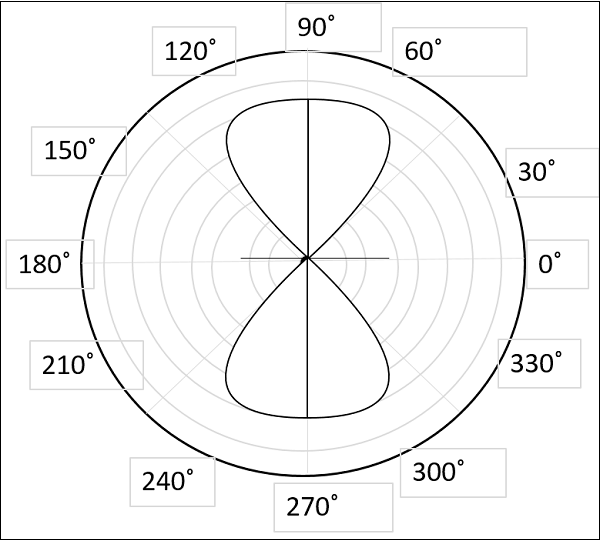The above figure shows the radiation pattern for normal mode of radiation in helical antenna.

It depends upon the values of diameter of helix, D and its turn spacing, S. Drawbacks of this mode of operation are low radiation efficiency and narrow bandwidth. Hence, it is hardly used.

### Axial mode

In axial mode of radiation, the radiation is in the end-fire direction along the helical axis and the waves are circularly or nearly circularly polarized. This mode of operation is obtained by raising the circumference to the order of one wavelength (λ) and spacing of approximately λ/4. The radiation pattern is broad and directional along the axial beam producing minor lobes at oblique angles.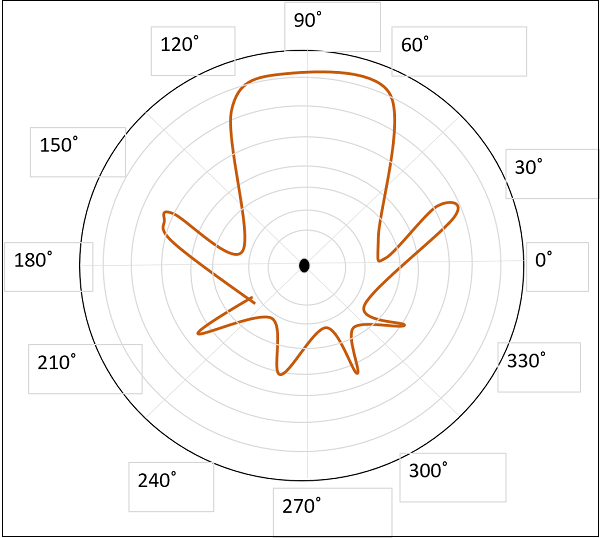The figure shows the radiation pattern for axial mode of radiation in helical antenna.

If this antenna is designed for right-handed circularly polarized waves, then it will not receive left-handed circularly polarized waves and vice versa. This mode of operation is generated with great ease and is more practically used.

The following are the advantages of Helical antenna −

• Simple design
• Highest directivity
• Wider bandwidth
• Can achieve circular polarization
• Can be used at HF & VHF bands also

The following are the disadvantages of Helical antenna −

• Antenna is larger and requires more space
• Efficiency decreases with number of turns

### Applications

The following are the applications of Helical antenna −

• A single helical antenna or its array is used to transmit and receive VHF signals
• Frequently used for satellite and space probe communications
• Used for telemetry links with ballastic missiles and satellites at Earth stations
• Used to establish communications between the moon and the Earth

# Antenna Theory – Aperture

An Antenna with an aperture at the end can be termed as an Aperture antenna. Waveguide is an example of aperture antenna. The edge of a transmission line when terminated with an opening, radiates energy. This opening which is an aperture, makes it an Aperture antenna.

The main types of aperture antennas are −

• Wave guide antenna
• Horn antenna
• Slot antenna

Let us now have a look at these types of aperture antennas.

## Waveguide Antenna

Waveguide is capable of radiating energy when excited at one end and opened at the other end. The radiation in wave guide is greater than a two-wire transmission line.

### Frequency Range

The operational frequency range of a wave guide is around 300MHz to 300GHz. This antenna works in UHF and EHF frequency ranges. The following image shows a waveguide.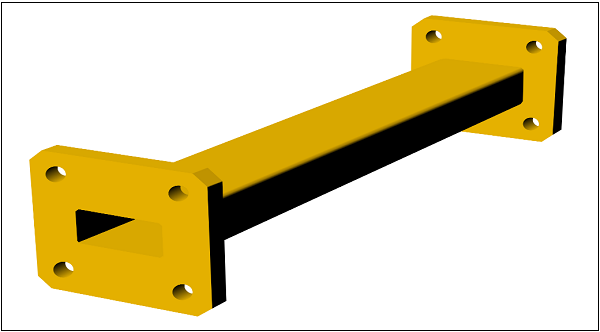This waveguide with terminated end, acts as an antenna. But only a small portion of the energy is radiated while a large portion of it gets reflected back in the open circuit. It means VSWR (voltage standing wave ratio, discussed in basic parameters chapter) value increases. The diffraction around the waveguide provides poor radiation and non-directive radiation pattern.

The radiation of waveguide antenna is poor and the pattern is non-directive, which means omni-directional. An omni-directional pattern is the one which has no certain directivity but radiates in all directions, hence it is called as non-directive radiation pattern.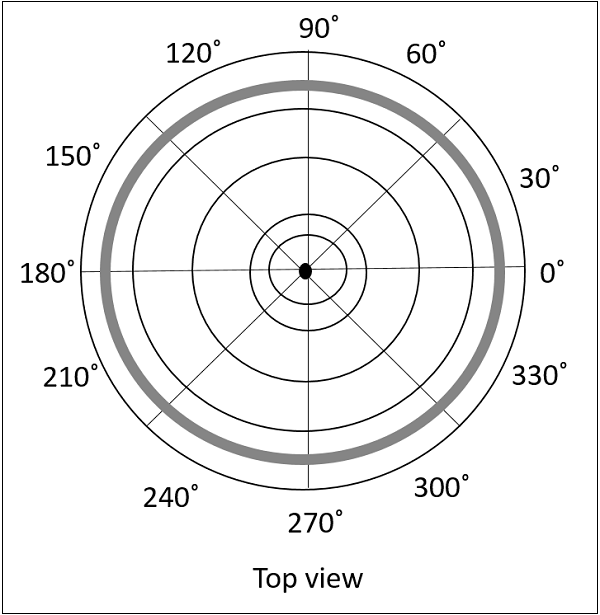The above figure shows a top section view of an omni-directional pattern, which is also called as non-directional pattern. The two-dimensional view is a figure-of-eight pattern, as we already know.

The following are the advantages of Aperture antenna −

• Radiation is greater than two-wire transmission line

The following are the disadvantages of Aperture antenna −

• VSWR increases

### Applications

The following are the applications of Aperture antenna −

• Micro wave applications

The waveguide antenna has to be further modified to achieve better performance, which results in the formation of Horn antenna.

# Antenna Theory – Horn

To improve the radiation efficiency and directivity of the beam, the wave guide should be provided with an extended aperture to make the abrupt discontinuity of the wave into a gradual transformation. So that all the energy in the forward direction gets radiated. This can be termed as Flaring. Now, this can be done using a horn antenna.

### Frequency Range

The operational frequency range of a horn antenna is around 300MHz to 30GHz. This antenna works in UHF and SHF frequency ranges.

## Construction & Working of Horn Antenna

The energy of the beam when slowly transform into radiation, the losses are reduced and the focussing of the beam improves. A Horn antenna may be considered as a flared out wave guide, by which the directivity is improved and the diffraction is reduced.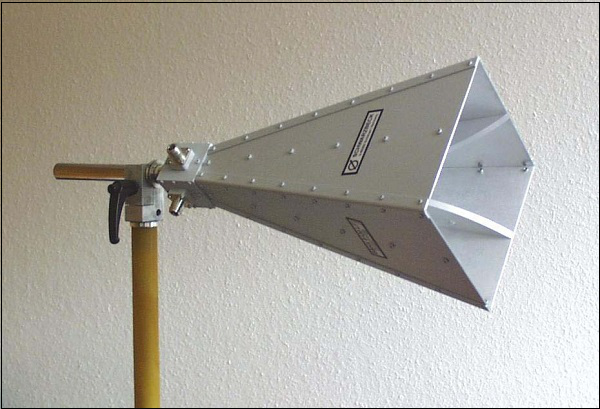The above image shows the model of a horn antenna. The flaring of the horn is clearly shown. There are several horn configurations out of which, three configurations are most commonly used.

### Sectoral horn

This type of horn antenna, flares out in only one direction. Flaring in the direction of Electric vector produces the sectorial E-plane horn. Similarly, flaring in the direction of Magnetic vector, produces the sectorial H-plane horn.

### Pyramidal horn

This type of horn antenna has flaring on both sides. If flaring is done on both the E & H walls of a rectangular waveguide, then pyramidal horn antenna is produced. This antenna has the shape of a truncated pyramid.

### Conical horn

When the walls of a circular wave guide are flared, it is known as a conical horn. This is a logical termination of a circular wave guide.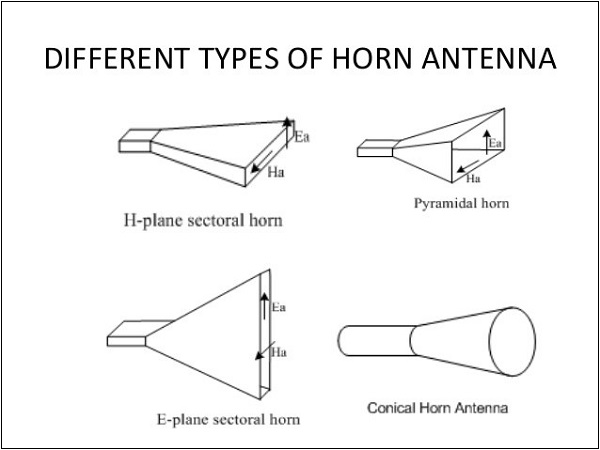The above figures show the types of horn configurations, which were discussed earlier.

Flaring helps to match the antenna impedance with the free space impedance for better radiation. It avoids standing wave ratio and provides greater directivity and narrower beam width. The flared wave guide can be technically termed as Electromagnetic Horn Radiator.

Flare angle, Φ of the horn antenna is an important factor to be considered. If this is too small, then the resulting wave will be spherical instead of plane and the radiated beam will not be directive. Hence, the flare angle should have an optimum value and is closely related to its length.

### Combinations

Horn antennas, may also be combined with parabolic reflector antennas to form special type of horn antennas. These are −

• Cass-horn antenna
• Hog-horn or triply folded horn reflector

In Cass-horn antenna, radio waves are collected by the large bottom surface, which is parabolically curved and reflected upward at 45° angle. After hitting top surface, they are reflected to the focal point. The gain and beam width of these are just like parabolic reflectors.

In hog-horn antenna, a parabolic cylinder is joined to pyramidal horn, where the beam reaches apex of the horn. It forms a low-noise microwave antenna. The main advantage of hog-horn antenna is that its receiving point does not move, though the antenna is rotated about its axis.

The radiation pattern of a horn antenna is a Spherical Wave front. The following figure shows the radiation pattern of horn antenna. The wave radiates from the aperture, minimizing the diffraction of waves. The flaring keeps the beam focused. The radiated beam has high directivity.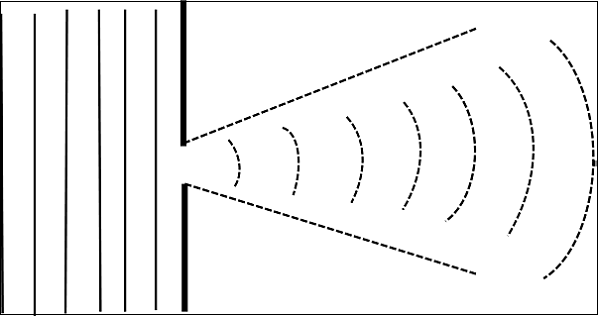The following are the advantages of Horn antenna −

• Small minor lobes are formed
• Impedance matching is good
• Greater directivity
• Narrower beam width
• Standing waves are avoided

The following are the disadvantages of Horn antenna −

• Designing of flare angle, decides the directivity
• Flare angle and length of the flare should not be very small

### Applications

The following are the applications of Horn antenna −

• Used for astronomical studies
• Used in microwave applications

# Antenna Theory – Slot

Slot Antenna is an example of Aperture antenna. A rectangular slot is made on the conducting sheet. These slot antennas can be formed by simply making a cut on the surface, where they are mounted on.

### Frequency Range

The frequency range used for the application of Slot antenna is 300 MHz to 30 GHz. It works in UHF and SHF frequency ranges.

## Construction & Working of Slot Antennas

The use of slot antennas is well understood through its working principle. Let us have a look at the structure of a slot antenna.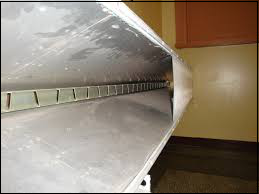When an infinite conducting sheet is made a rectangular cut and the fields are excited in the aperture (which is called as a slot), it is termed as Slot antenna. This can be understood by observing the image of a slot antenna. The following image shows the model of a Slot antenna.

The working of Slot Antenna can be easily understood through Babinet’s principle of optics. This concept gives an introduction to the slot antennas.

## Babinet’s Principle

Babinet’s principle states that- “When the field behind a screen with an opening is added to the field of a complementary structure, the sum is equal to the field when there is no screen”.

Case 1 − Consider a light source and a conducting plane (field) with an aperture before a screen. The light does not pass through the opaque area, but passes through the aperture.

Case 2 − Consider the light source and a conducting plane of the size of the aperture in the previous case, being held against the screen. The light does not pass through the plane but through the remaining portion.

Case 3 − Combine these two conducting planes of both the cases and put before the light source. The screen is not placed to observe the resultant combination. The effect of screen gets nullified.

## Working of Slot Antenna

This principle of optics is applied to electromagnetic waves for the wave to get radiated. It is true that when a HF field exists across a narrow slot in a conducting plane, the energy is radiated.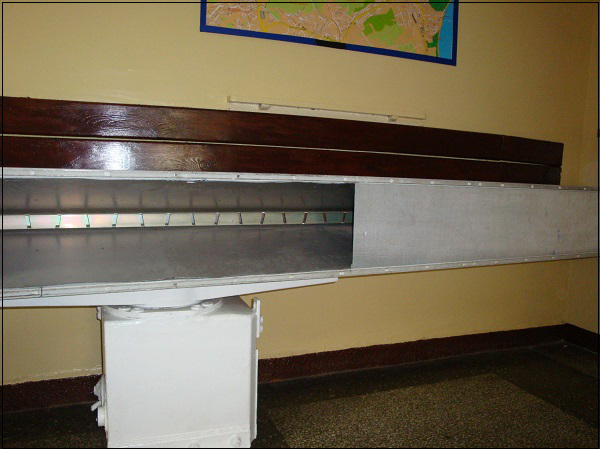The image shows a slot antenna, which explains well about its working.

Consider an infinite plane conducting screen is taken and pierced with apertures of desired shape and size and this will be the screen of slot antenna. Another screen is considered interchanging the places of aperture and screen area which is the complementary screen.

These two screens are said to be complementary as they result in complete infinte metal screen. Now, this becomes the slot antenna. The terminal impedance is quite desirable for the radiation.

The radiation pattern of the Slot antenna is Omni-directional, just like a half-wave dipole antenna. Take a look at the following illustration. It shows the radiation pattern of Slot antenna drawn in Horizontal and Vertical planes respectively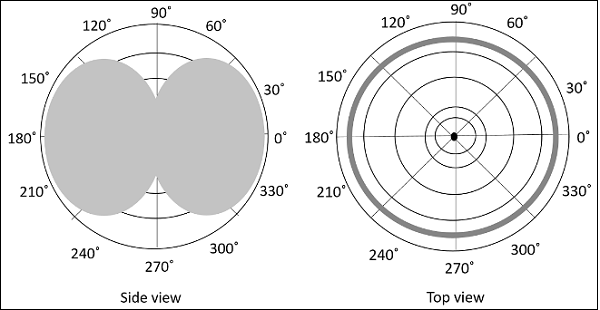The following are the advantages of Slot antenna −

• It can be fabricated and concealed within metallic objects
• It can provide covert communications with a small transmitter

The following are the disadvantages of Slot antenna −

• Higher cross-polarization levels

### Applications

The following are the applications of Slot antenna −

• Used as an array fed by a wave guide

# Antenna Theory – Micro Strip

Micro strip antennas are low-profile antennas. A metal patch mounted at a ground level with a di-electric material in-between constitutes a Micro strip or Patch Antenna. These are very low size antennas having low radiation.

### Frequency Range

The patch antennas are popular for low profile applications at frequencies above 100MHz.

## Construction & Working of Micro strip Antennas

Micro strip antenna consists of a very thin metallic strip placed on a ground plane with a di-electric material in-between. The radiating element and feed lines are placed by the process of photo-etching on the di-electric material. Usually, the patch or micro-strip is choosen to be square, circular or rectangular in shape for the ease of analysis and fabrication. The following image shows a micro-strip or patch antenna.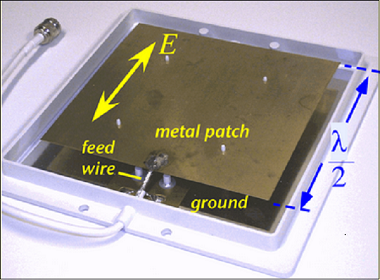The length of the metal patch is λ/2. When the antenna is excited, the waves generated within the di-electric undergo reflections and the energyis radiated from the edges of the metal patch,which is very low.

The radiation pattern of microstrip or patch antenna is broad. It has low radiation power and narrow frequency bandwidth.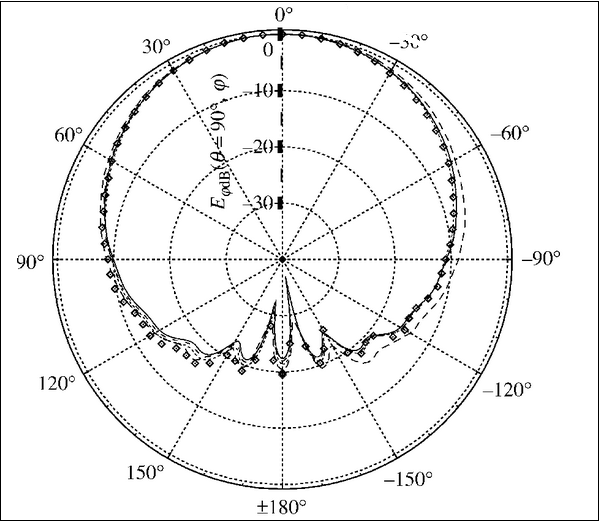The radiation pattern of a microstrip or patch antenna is shown above. It has lesser directivity. To have a greater directivity, an array can be formed by using these patch antennas.

The following are the advantages of Micro strip antenna −

• Lighteweight
• Low cost
• Ease of installation

The following are the disadvantages of Micro strip antenna −

• Narrow frequency bandwidth

### Applications

The following are the applications of Micro strip antenna −

• Used in Space craft applications
• Used in Air craft applications
• Used in Low profile antenna applications

# Antenna Theory – Lens

The antennas, which we have discussed till now, used the plane surface. The lens antennas use the curved surface for both transmission and reception. Lens antennas are made up of glass, where the converging and diverging properties of lens are followed. The lens antennas are used for higher frequency applications.

### Frequency Range

The frequency range of usage of lens antenna starts at 1000 MHz but its use is greater at 3000 MHz and above.

To have a better understanding of the lens antenna, the working principle of a lens has to be known. A normal glass lens works on the principle of refraction.

## Construction & Working of Lens Antenna

If a light source is assumed to be present at a focal point of a lens, which is at a focal distance from the lens, then the rays get through the lens as collimated or parallel rays on the plane wavefront.

The rays that pass through the centre of the lens are less refracted than the rays that pass through the edges of the lens. All of the rays are sent in parallel to the plane wave front. This phenomenon of lens is called as divergence.

The same procedure gets reversed if a light beam is sent from right side to left of the same lens. Then the beam gets refracted and meets at a point called focal point, at a focal distance from the lens. This phenomenon is called convergence.

The same can be better understood by observing the following diagram −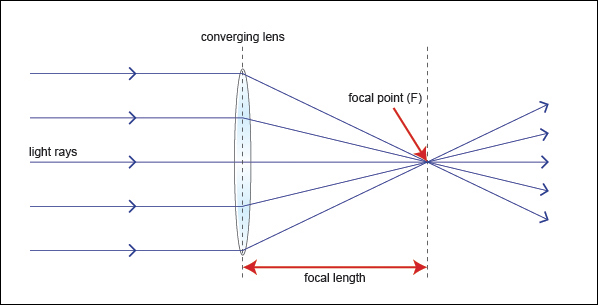The ray diagram represents the focal point and focal length from the source to the lens. The parallel rays obtained are also called as collimated rays.

In the above figure, the source at the focal point, at a focal distance from the lens, gets collimated in the plane wave front. This phenomenon can be reversed which means the light if sent from the left side, gets converged at the right side of the lens.

It is because of this reciprocity, the lens can be used as an antenna, as the same phenomenon helps in utilizing the same antenna for both transmission and reception.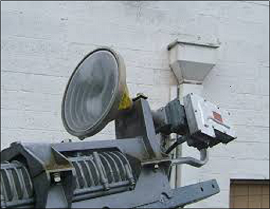The image of the model of a lens antenna is shown.

To achieve the focusing properties at higher frequencies, the refractive index should be less than unity. Whatever may be the refractive index, the purpose of lens is to straighten the waveform. Based on this, the E-plane and H-plane lens are developed, which also delay or speed up the wave front.

## Types of Lens Antennas

The following types of Lens Antennas are available −

• Di-electric lens or H-plane metal plate lens or Delay lens (Travelling waves are delayed by lens media)
• E-plane metal plate lens
• Non-metallic di-electric type lens
• Metallic or artificial dielectric type of lens

The following are the advantages of Lens antenna −

• In lens antennas, feed and feed support, do not obstruct the aperture.
• It has greater design tolerance.
• Larger amount of wave, than a parabolic reflector, can be handled.
• Beam can be moved angularly with espect to the axis.

The following are the disadvantages of Lens antenna −

• Lenses are heavy and bulky, especially at lower frequencies
• Complexity in design
• Costlier compared to reflectors, for the same specifications

### Applications

The following are the applications of Lens antenna −

• Used as wide band antenna
• Especially used for Microwave frequency applications

The converging properties of lens antennas can be used for developing higher level of antennas known as Parabolic reflector antennas, which are widely used in satellite communications. We will discuss about them in the next chapter.

# Antenna Theory – Parabolic Reflector

Parabolic Reflectors are Microwave antennas. For better understanding of these antennas, the concept of parabolic reflector has to be discussed.

### Frequency Range

The frequency range used for the application of Parabolic reflector antennas is above 1MHz. These antennas are widely used for radio and wireless applications.

## Principle of Operation

The standard definition of a parabola is – Locus of a point, which moves in such a way that its distance from the fixed point (called focus) plus its distance from a straight line (called directrix) is constant.

The following figure shows the geometry of parabolic reflector. The point F is the focus (feed is given) and V is the vertex. The line joining F and V is the axis of symmetry. PQ are the reflected rays where L represents the line directrix on which the reflected points lie (to say that they are being collinear). Hence, as per the above definition, the distance between F and L lie constant with respect to the waves being focussed.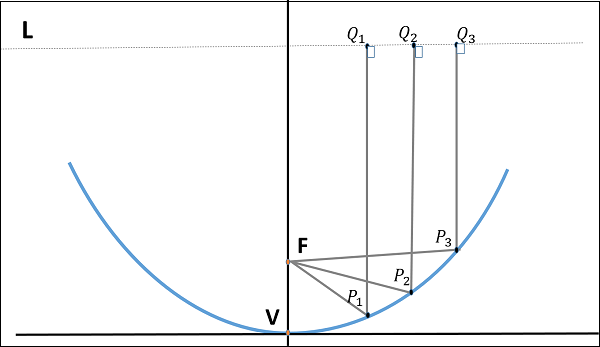The reflected wave forms a colllimated wave front, out of the parabolic shape. The ratio of focal length to aperture size (ie., f/D) known as “f over D ratio” is an important parameter of parabolic reflector. Its value varies from 0.25 to 0.50.

The law of reflection states that the angle of incidence and the angle of reflection are equal. This law when used along with a parabola, helps the beam focus. The shape of the

parabola when used for the purpose of reflection of waves, exhibits some properties of the parabola, which are helpful for building an antenna, using the waves reflected.

### Properties of Parabola

• All the waves originating from focus, reflects back to the parabolic axis. Hence, all the waves reaching the aperture are in phase.
• As the waves are in phase, the beam of radiation along the parabolic axis will be strong and concentrated.

Following these points, the parabolic reflectors help in producing high directivity with narrower beam width.

## Construction & Working of a Parabolic Reflector

If a Parabolic Reflector antenna is used for transmitting a signal, the signal from the feed, comes out of a dipole or a horn antenna, to focus the wave on to the parabola. It means that, the waves come out of the focal point and strike the Paraboloidal reflector. This wave now gets reflected as collimated wave front, as discussed previously, to get transmitted.

The same antenna is used as a receiver. When the electromagnetic wave hits the shape of the parabola, the wave gets reflected onto the feed point. The dipole or the horn antenna, which acts as the receiver antenna at its feed, receives this signal, to convert it into electric signal and forwards it to the receiver circuitry.

The following image shows a Parabolic Reflector Antenna.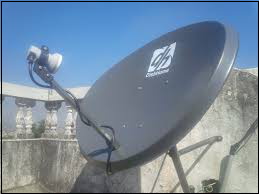The gain of the paraboloid is a function of aperture ratio (D/λ). The Effective Radiated Power (ERP) of an antenna is the multiplication of the input power fed to the antenna and its power gain.

Usually a wave guide horn antenna is used as a feed radiator for the paraboloid reflector antenna. Along with this technique, we have another type of feed given to the paraboloid reflector antenna, called as Cassegrain feed.

## Cassegrain Feed

Casse grain is another type of feed given to the reflector antenna. In this type, the feed is located at the vertex of the paraboloid, unlike in the parabolic reflector. A convex shaped reflector, which acts as a hyperboloid is placed opposite to the feed of the antenna. It is also known as secondary hyperboloid reflector or sub-reflector. It is placed such that its one of the foci coincides with the focus of the paraboloid. Thus, the wave gets reflected twice.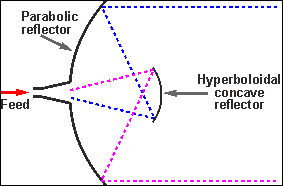The above figure shows the working model of cassegrain feed.

## Working of a Cassegrain Antenna

When the antenna acts as a transmitting antenna, the energy from the feed radiates through a horn antenna onto the hyperboloid concave reflector, which again reflects back on to the parabolic reflector. The signal gets reflected into the space from there. Hence, wastage of power is controlled and the directivity gets improved.

When the same antenna is used for reception, the electromagnetic waves strike the reflector, gets reflected on to the concave hyperboloid and from there, it reaches to the feed. A wave guide horn antenna presents there to receive this signal and sends to the receiver circuitry for amplification.

Take a look at the following image. It shows a paraboloid reflector with cassegrain feed.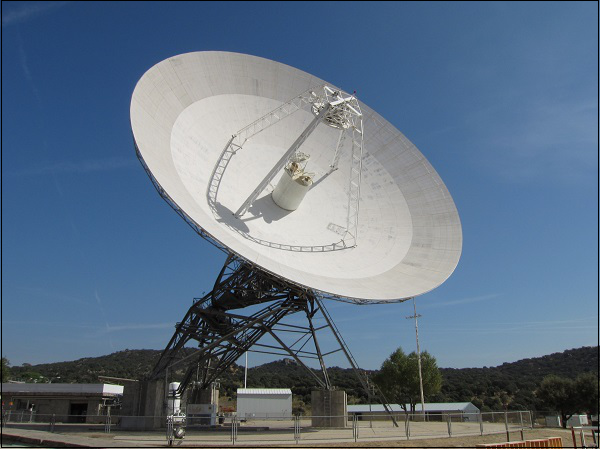The following are the advantages of Parabolic reflector antenna −

• Reduction of minor lobes
• Wastage of power is reduced
• Equivalent focal length is achieved
• Feed can be placed in any location, according to our convenience
• Adjustment of beam (narrowing or widening) is done by adjusting the reflecting surfaces

The following is the disadvantage of a Parabolic reflector antenna −

• Some of the power that gets reflected from the parabolic reflector is obstructed. This becomes a problem with small dimension paraboloid.

### Applications

The following are the applications of Parabolic reflector antenna −

• The cassegrain feed parabolic reflector is mainly used in satellite communications.
• Also used in wireless telecommunication systems.

Let us look at the other type of feed called as Gregorian feed for the parabolic reflectors.

## Gregorian Feed

This is another type of feed used. A pair of certain configurations are there, where the feed beamwidth is progressively increased while antenna dimensions are held fixed. Such a type of feed is known as Gregorian feed. Here, the convex shaped hyperboloid of casssegrain is replaced with a concave shaped paraboloid reflector, which is of course, smaller in size

These Gregorian feed type reflectors can be used in four ways −

• Gregorian systems using reflector ellipsoidal sub-reflector at foci F1.
• Gregorian systems using reflector ellipsoidal sub-reflector at foci F2.
• Cassegrain systems using hyperboloid sub-reflector (convex).
• Cassegrain systems using hyperboloid sub-reflector (concave but the feed being very near to it.)

These are all just to mention because they are not popular and are not widely used. They have got their limitations.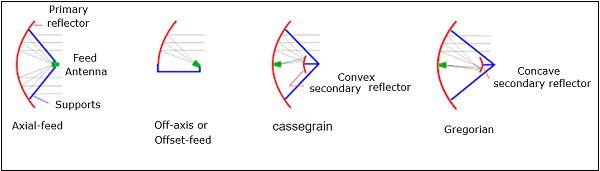The figure clearly depicts the working pattern of all the types of reflectors. There are other types of paraboloid Reflectors such as −

• Cut- paraboloid
• Parabolic cylinder
• Pill-box paraboloid

However, all of them are seldom used because of the limitations and disavantages they have in their working conditions.

Hence, of all the types of reflector antennas, the simple parabolic reflectors and the cassegrain feed parabolic reflectors are the most commonly used ones.

# Antenna Theory – Antenna Arrays

An antenna, when individually can radiate an amount of energy, in a particular direction, resulting in better transmission, how it would be if few more elements are added it, to produce more efficient output. It is exactly this idea, which led to the invention of Antenna arrays.

An antenna array can be better understood by observing the following images. Observe how the antenna arrays are connected.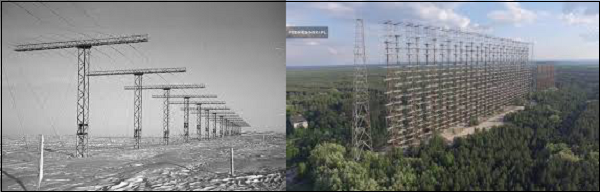An antenna array is a radiating system, which consists of individual radiators and elements. Each of this radiator, while functioning has its own induction field. The elements are placed so closely that each one lies in the neighbouring one’s induction field. Therefore, the radiation pattern produced by them, would be the vector sum of the individual ones. The following image shows another example of an antenna array.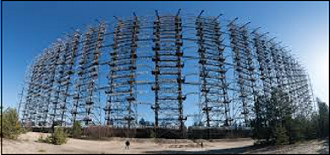The spacing between the elements and the length of the elements according to the wavelength are also to be kept in mind while designing these antennas.

The antennas radiate individually and while in array, the radiation of all the elements sum up, to form the radiation beam, which has high gain, high directivity and better performance, with minimum losses.

The following are the advantages of using antenna arrays −

• The signal strength increases
• High directivity is obtained
• Minor lobes are reduced much
• High Signal-to-noise ratio is achieved
• High gain is obtained
• Power wastage is reduced
• Better performance is obtained

The following are the disadvantages of array antennas −

• Resistive losses are increased
• Mounting and maintenance is difficult
• Huge external space is required

### Applications

The following are the applications of array antennas −

• Used in satellite communications
• Used in wireless communications
• Used in military radar communications
• Used in the astronomical study

## Types of Arrays

The basic types of arrays are −

• Collinear array
• End fire array
• Parasitic array
• Yagi-Uda array
• Log-peroidic array
• Turnstile array
• Super-turnstile array

We will discuss these arrays in the coming chapters.

# Antenna Theory – Collinear Array

Collinear array consists of two or more half-wave dipoles, which are placed end to end. These antennas are placed on a common line or axis, being parallel or collinear.

The maximum radiation in these arrays is broad side and perpendicular to the line of array. These arrays are also called as broad cast or Omni-directional arrays.

### Frequency range

The frequency range in which the collinear array antennas operate is around 30 MHz to 3GHz which belong to the VHF and UHF bands.

## Construction of Array

These collinear arrays are uni-directional antennas having high gain. The main purpose of this array is to increase the power radiated and to provide high directional beam, by avoiding power loss in other directions.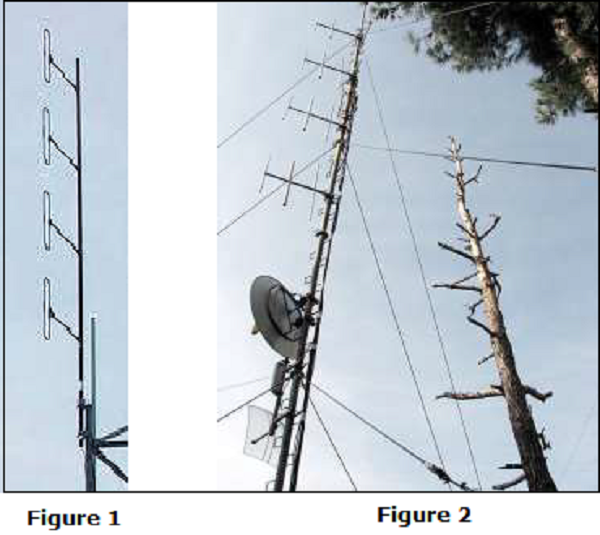The above images show the pictures of collinear arrays. In figure 1, it is seen that collinear array is formed using folded dipoles, while in figure 2, the collinear array is formed by normal dipoles. Both types are half-wave dipoles used commonly.

The radiation pattern of these collinear arrays is similar to that of a single dipole, but the array pattern of increasing number of dipoles, makes the difference.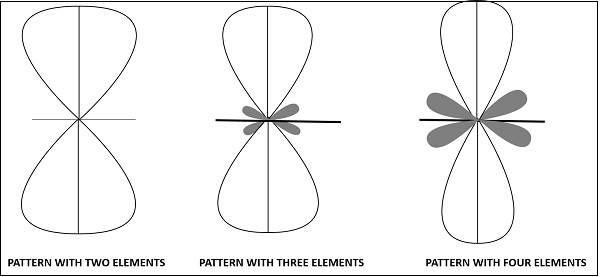The radiation pattern of collinear array when made using two elements, three elements and four elements respectively are shown in the figure given above.

The broad side array also has the same pattern, in which the direction of maximum radiation is perpendicular to the line of antenna.

The following are the advantages of collinear array antennas −

• Use of array reduces the broad ends and increases the directivity
• Minor lobes are minimised
• Wastage of power is reduced

The following are the disadvantages of collinear array antennas −

• Displacement of these antennas is a difficult task
• Used only in outdoor areas

### Applications

The following are the applications of collinear array antennas −

• Used for VHF and UHF bands
• Used in two-way communications
• Used also for broadcasting purposes

# Antenna Theory – Broad-side Array

The antenna array in its simplest form, having a number of elements of equal size, equally spaced along a straight line or axis, forming collinear points, with all dipoles in the same phase, from the same source together form the broad side array.

### Frequency range

The frequency range, in which the collinear array antennas operate is around 30 MHz to 3GHz which belong to the VHF and UHF bands.

## Construction & Working of Broad-side Array

According to the standard definition, “An arrangement in which the principal direction of radiation is perpendicular to the array axis and also to the plane containing the array element” is termed as the broad side array. Hence, the radiation pattern of the antenna is perpendicular to the axis on which the array exists.

The following diagram shows the broad side array, in front view and side view, respectively.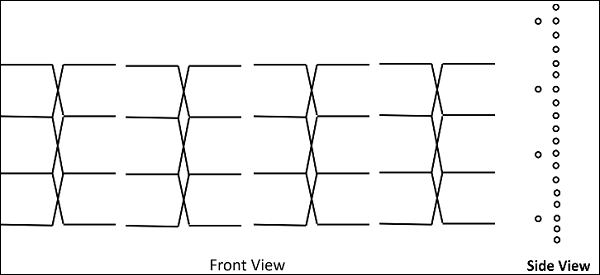The broad side array is strongly directional at right angles to the plane of the array. However, the radiation in the plane will be very less because of the cancellation in the direction joining the center.

The figure of broad side array with λ/4 spacing is shown below.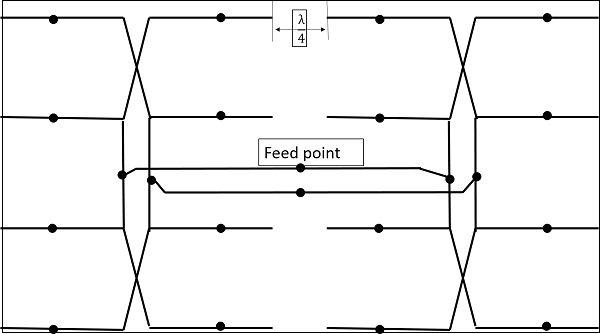Typical antenna lengths in the broad side array are from 2 to 10 wavelengths. Typical spacings are λ/2 or λ. The feed points of the dipoles are joined as shown in the figure.

The radiation pattern of this antenna is bi-directional and right angles to the plane. The beam is very narrow with high gain.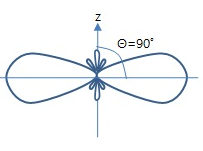The above figure shows the radiation pattern of the broad side array. The beam is a bit wider and minor lobes are much reduced in this.

# Antenna Theory – End-fire Array

The physical arrangement of end-fire array is same as that of the broad side array. The magnitude of currents in each element is same, but there is a phase difference between these currents. This induction of energy differs in each element, which can be understood by the following diagram.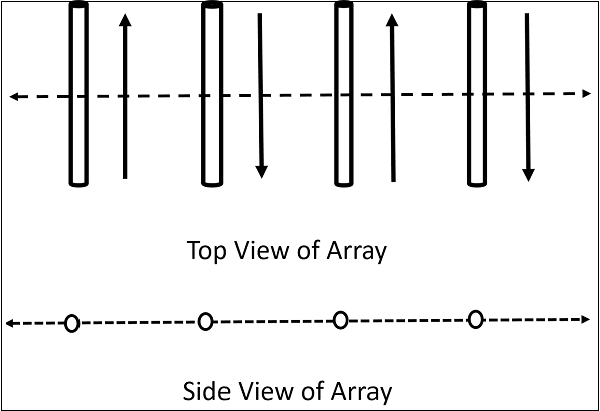The above figure shows the end-fire array in top and side views respectively.

There is no radiation in the right angles to the plane of the array because of cancellation. The first and third elements are fed out of phase and therefore cancel each other’s radiation. Similarly, second and fourth are fed out of phase, to get cancelled.

The usual dipole spacing will be λ/4 or 3λ/4. This arrangement not only helps to avoid the radiation perpendicular to the antenna plane, but also helps the radiated energy get diverted to the direction of radiation of the whole array. Hence, the minor lobes are avoided and the directivity is increased. The beam becomes narrower with the increased elements.

The Radiation pattern of end-fire array is uni-directional. A major lobe occurs at one end, where maximum radiation is present, while the minor lobes represent the losses.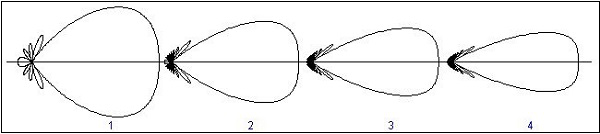The figure explains the radiation pattern of an end-fire array. Figure 1 is the radiation pattern for a single array, while figures 2, 3, and 4 represent the radiation pattern for multiple arrays.

## End-fire Array Vs Broad Side Array

We have studied both the arrays. Let us try to compare the end-fire and broad side arrays, along with their characteristics.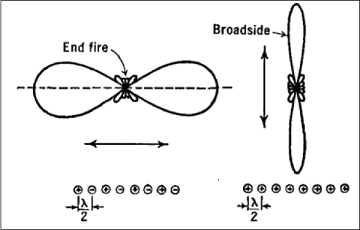The figure illustrates the radiation pattern of end-fire array and broad side array.

• Both, the end fire array and broad side array, are linear and are resonant, as they consist of resonant elements.
• Due to resonance, both the arrays display narrower beam and high directivity.
• Both of these arrays are used in transmission purposes.
• Neither of them is used for reception, because the necessity of covering a range of frequencies is needed for any kind of reception.

# Antenna Theory – Parasitic Array

The antenna arrays as seen above, are used for the improvement of gain and directivity.

parasitic element is an element, which depends on other’s feed. It does not have its own feed. Hence, in this type of arrays we employ such elements, which help in increasing the radiation indirectly.

These parasitic elements are not directly connected to the feed.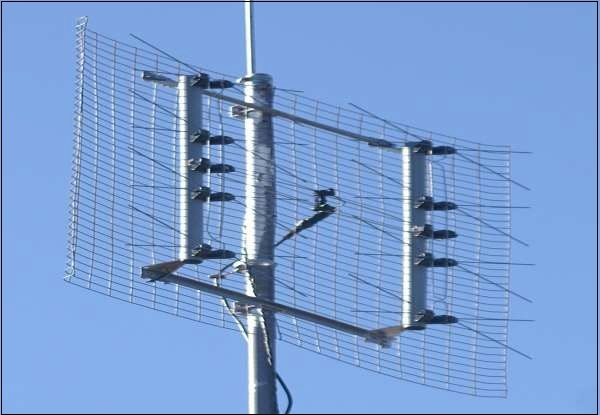The above image shows an example of a parasitic array. The mesh structure seen in the picture, is nothing but a set of reflectors. These reflectors are not electrically connected. They increase the signal strength by increasing the directivity of the beam.

## Construction & Working of Parasitic Array

Let us look at the important parts of a Parasitic array and how they work.

The main parts are −

• Driven element
• Parasitic elements
• Reflector
• Director
• Boom

### Driven element

The antennas radiate individually and while in array, the radiation of all the elements sum up to form the radiation beam. All the elements of the array need not be connected to the feed. The dipole that is connected to the feed is known as a driven element.

### Parasitic Elements

The elements, which are added do not possess an electrical connection between them to the driven element or the feed. They are positioned so that they lie in the induction field of the driven element. Hence, they are known as parasitic elements.

Reflector

If one of the parasitic element, which is 5% longer than driven element, is placed close to the driven element is longer, then it acts as a concave mirror, which reflects the energy in the direction of the radiation pattern rather than its own direction and hence is known as a reflector.

Director

A parasitic element, which is 5% shorter than the driven element, from which it receives energy, tends to increase radiation in its own direction and therefore, behaves like convergent convex lens. This element is called as a director. A number of directors are placed to increase the directivity.

### Boom

The element on which all these are placed is callled a boom. It is a non-metallic structure which provides insulation, so that there will not be any short circuit between the other elements of the array.

These are all the main elements, which contribute the radiation. This can be better understood with the help of a diagram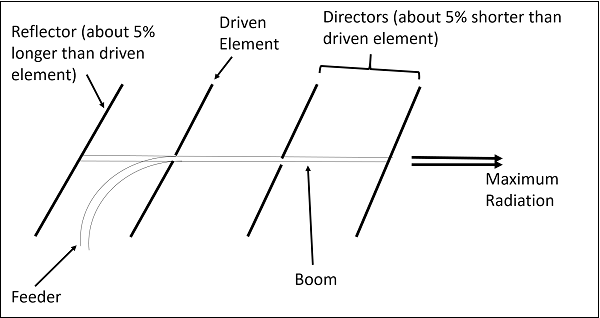The image shown above is that of a parasitic array, which shows the parts of parsitic array such as the driven element, the directors and the reflector. The feed is given through the feeder.

The arrays are used at frequencies ranging from 2MHz to several GHz. These are especially used to get high directivity, and better forward gain with a uni-directional. The most common example of this type of array is the Yagi-Uda antenna. Quad antenna may also be quoted as another example.

# Antenna Theory – Yagi-Uda Antenna

Yagi-Uda antenna is the most commonly used type of antenna for TV reception over the last few decades. It is the most popular and easy-to-use type of antenna with better performance, which is famous for its high gain and directivity

Frequency range

The frequency range in which the Yagi-Uda antennas operate is around 30 MHz to 3GHz which belong to the VHF and UHF bands.

## Construction of Yagi-Uda Antenna

A Yagi-Uda antenna was seen on top of almost every house during the past decades. The parasitic elements and the dipole together form this Yagi-Uda antenna.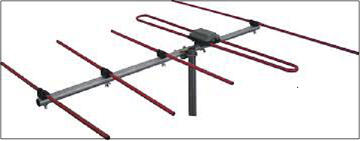The figure shows a Yagi-Uda antenna. It is seen that there are many directors placed to increase the directivity of the antenna. The feeder is the folded dipole. The reflector is the lengthy element, which is at the end of the structure.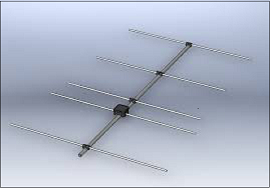The figure depicts a clear form of the Yagi-Uda antenna. The center rod like structure on which the elements are mounted is called as boom. The element to which a thick black head is connected is the driven element to which the transmission line is connected internally, through that black stud. The single element present at the back of the driven element is the reflector, which reflects all the energy towards the direction of the radiation pattern. The other elements, before the driven element, are the directors, which direct the beam towards the desired angle.

### Designing

For this antenna to be designed, the following design specifications should be followed.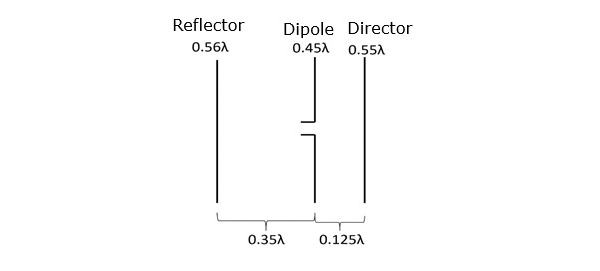They are −

ELEMENT SPECIFICATION
Length of the Driven Element 0.458λ to 0.5λ
Length of the Reflector 0.55λ to 0.58λ
Length of the Director 1 0.45λ
Length of the Director 2 0.40λ
Length of the Director 3 0.35λ
Spacing between Directors 0.2λ
Reflector to dipole spacing 0.35λ
Dipole to Director spacing 0.125λ

If the specifications given above are followed, one can design an Yagi-Uda antenna.

The directional pattern of the Yagi-Uda antenna is highly directive as shown in the figure given below.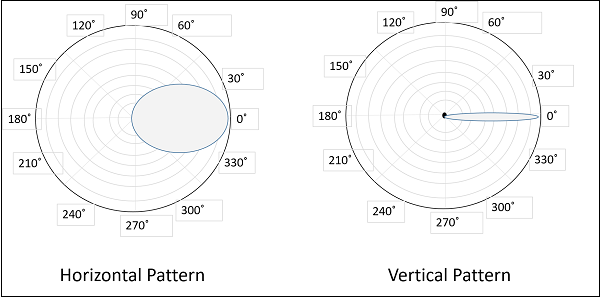The minor lobes are suppressed and the directivity of the major lobe is increased by the addition of directors to the antenna.

The following are the advantages of Yagi-Uda antennas −

• High gain is achieved.
• High directivity is achieved.
• Ease of handling and maintenance.
• Less amount of power is wasted.

The following are the disadvantages of Yagi-Uda antennas −

• Prone to noise.
• Prone to atmospheric effects.

### Applications

The following are the applications of Yagi-Uda antennas −

• Mostly used for TV reception.
• Used where a single-frequency application is needed.

# Antenna Theory – Log-periodic Antenna

The Yagi-Uda antenna is mostly used for domestic purpose. However, for commercial purpose and to tune over a range of frequencies, we need to have another antenna known as the Log-periodic antenna. A Log-periodic antenna is that whose impedance is a logarithamically periodic function of frequency.

### Frequency range

The frequency range, in which the log-periodic antennas operate is around 30 MHz to 3GHz which belong to the VHF and UHF bands.

## Construction & Working of Log-periodic Antenna

The construction and operation of a log-periodic antenna is similar to that of a Yagi-Uda antenna. The main advantage of this antenna is that it exhibits constant characteristics over a desired frequency range of operation. It has the same radiation resistance and therefore the same SWR. The gain and front-to-back ratio are also the same.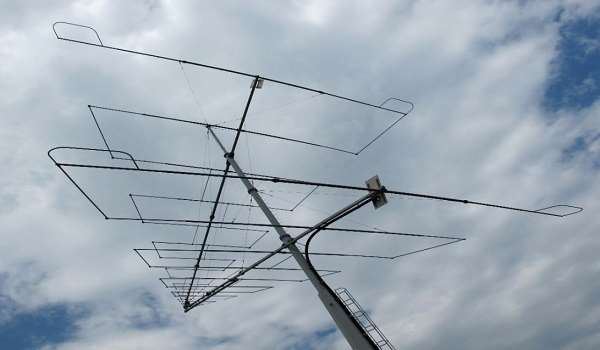The image shows a log-periodic antenna.

With the change in operation frequency, the active region shifts among the elements and hence all the elements will not be active only on a single frequency. This is its special characteristic.

There are several type of log-periodic antennas such as the planar, trapezoidal, zig-zag, V-type, slot and the dipole. The mostly used one is log-periodic dipole array, in short, LPDA.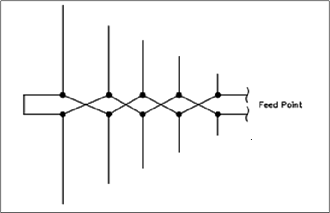The diagram of log-periodic array is given above.

The physical structure and electrical characteristics, when observed, are repetitive in nature. The array consists of dipoles of different lengths and spacing, which are fed from a two-wire transmission line. This line is transposed between each adjacent pair of dipoles.

The dipole lengths and separations are related by the formula −

R1R2=R2R3=R3R4=T=l1l2=l2l3=l3l4R1R2=R2R3=R3R4=T=l1l2=l2l3=l3l4

Where

• т is the design ratio and т<1
• R is the distance between the feed and the dipole
• l is the length of the dipole.

The directive gains obtained are low to moderate. The radiational patterns may be Unidirectional or Bi-directional.

The Radiation pattern of log-periodic antenna can be of uni-directional or bi-directional, depending upon the log periodic structures.

For uni-directional Log-periodic antenna, the radiation towards shorter element is of considerable amount, whereas in forward direction, it is small or zero.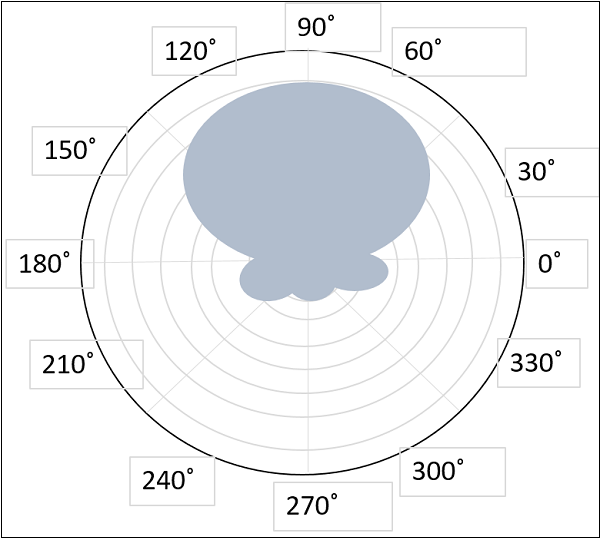The radiational pattern for uni-directional log-periodic antenna is given above.

For bi-directional Log-periodic antenna, the maximum radiation is in broad side, which is normal to the surface of the antenna.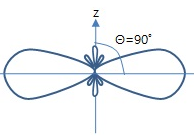The figure given above shows the radiational pattern for a bi-directional log-periodic antenna.

The following are the advantages of Log-periodic antennas −

• The antenna design is compact.
• Gain and radiation pattern are varied according to the requirements.

The following are the disadvantages of Log-periodic antennas −

• External mount.
• Installation cost is high.

### Applications

The following are the applications of Log-periodic antennas −

• Used for HF communications.
• Used for particular sort of TV receptions.
• Used for all round monitoring in higher frequency bands.

# Antenna Theory – Turnstile Antenna

The Turnstile antenna is another type of array antenna. The shape of this array symbolizes the turnstile, which is used at the entrances of few places. This antenna has a wide variety of military applications.

### Frequency range

The frequency range in which the turnstile antennas operate is around 30 MHz to 3GHz which belong to the VHF and UHF bands.

## Construction & Working of Turnstile Antenna

Two identical half-wave dipoles are placed at right angles to each other and are fed inphase. These dipoles are excited 90° out of phase with each other. Turnstile array can also be termed as crossed dipoles array.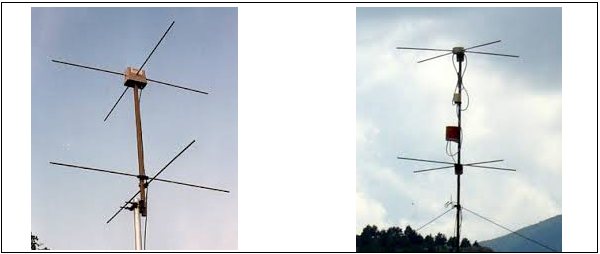The above images illustrate turnstile antennas.

To provide high directivity, several turnstiles may be stacked along a vertical axis, and are phased as shown in the figure given above. The polarization of these turnstile antennas depend upon their mode of operation.

The pair of such dipoles frequently stacked, is known as BAY. In the figures shown above, two bays are spaced half wavelength (λ/2) apart and the corresponding elements are fed in phase. The radiation produce by the combination of bays results in better directivity.

## Modes of Operation

The following are the modes of operation of a Turnstile antenna.

Normal mode

In Normal mode of operation, the antenna radiates horizontally polarized waves which are perpendicular to its axis.

Axial mode

In Axial mode of operation, the antenna radiates circularly polarized waves along its axis i.e. parallel to its axis.

For circular polarization, the transmitter radiating with right-circular polarization should have a receiver with same right-circular polarization and vice versa. If it is left-circular polarized one, unlike the transmitter, there will be a severe loss of gain.

## Super Turnstile Antenna

For a turnstile antenna, the radiation power is 3dB below the maximum radiation of a halfwave dipole radiating the same power. Therefore, to overcome this disadvantage, the Super-turnstile antenna is built.

The simple dipole elements in turnstile are replaced by four flat sheets in Super-turnstile. The design of Super-turnstile array is such that 1 to 8 bays can be constructed on a single mast. The other name for Super-turnstile antenna is the Batwing Antenna.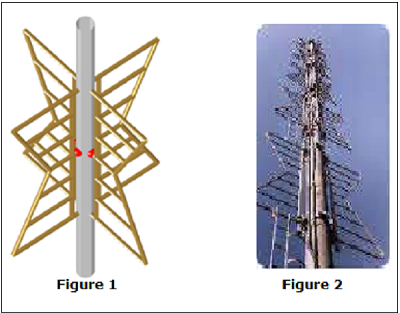The above images show super-turnstile antenna. Figure 1 shows the arrangement of superturnstile array with the red dots being the feed points. Figure 2 shows the stacked turnstile array used in satellite communications.

The radiation pattern will be similar to the radiation pattern of two super imposed dipoles. Though it is close to omni-directional pattern, it leaves a cloveleaf shaped pattern.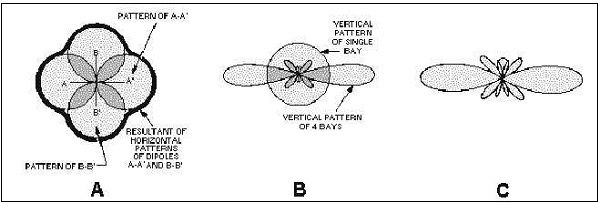The above figure shows the radiational pattern of a turnstile array. The typical figure-ofeight patterns were combined to produce a nearly circular pattern.

• Figure A shows the individual patterns being combined.
• Figure B shows the vertical pattern of single bay and also the combined pattern of four bays.
• Figure C shows the resultant combined pattern of four bays showing better directivity.

The following are the advantages of Turnstile antennas −

• High-gain is achieved by stacking
• Super-turnstile produces high-gain output
• Better directivity is achieved

The following is the disadvantage of Turnstile antennas −

• The radiation power is 3dB below the maximum radiation of a half wave dipole radiating the same power.

### Applications

The following are the applications of Turnstile antennas −

• Used for VHF communications
• Used for FM and TV broadcasting
• Used in military communications
• Used in satellite communications

# Antenna Theory – Spectrum & Transmission

In the Earth’s atmosphere, the propagation of wave depends not only on the properties of the wave, but also on environment effects and the layers of earth’s atmosphere. All of these have to be studied in order to form an idea of how a wave propagates in the environment.

Let us look at the frequency spectrum over which the signal transmission or reception takes place. Different types of antennas are manufactured depending upon the frequency range in which they are operated.

## Electromagnetic Spectrum

Wireless communication is based on the principle of broadcast and reception of electromagnetic waves. These waves can be characterized by their frequency (f) and their wavelength (λ) lambda.

A pictorial representation of the electromagnetic spectrum is given in the following figure.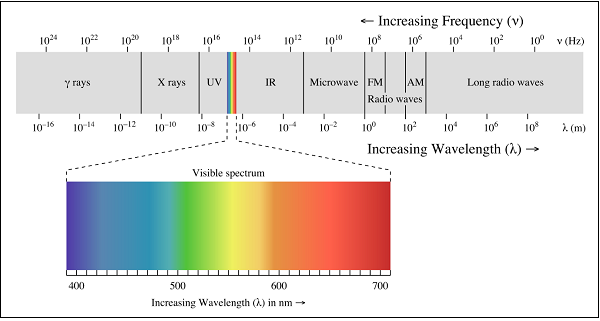### Low Frequency bands

Low Frequency bands comprise of the radio, microwave, infrared and visible portions of the spectrum. They can be used for information transmission by modulating the amplitude, frequency or phase of the waves.

### High Frequency bands

High Frequency bands comprise of X-rays and Gamma rays. Theoretically, these waves are better for information propagation. However, these waves are not used practically because of difficulty in modulation and the waves are harmful to living beings. In addition, high frequency waves do not propagate well through buildings.

## Frequency Bands and their Uses

The following table depicts the frequency bands and its uses −

Band Name Frequency Wavelength Applications
Extremely Low Frequency (ELF) 30 Hz to 300 Hz 10,000 to 1,000 KM Power line frequencies
Voice Frequency (VF) 300 Hz to 3 KHz 1,000 to 100 KM Telephone Communications
Very Low Frequency (VLF) 3 KHz to 30 KHz 100 to 10 KM Marine Communications
Low Frequency (LF) 30 KHz to 300 KHz 10 to 1 KM Marine Communications
Medium Frequency (MF) 300 KHz to 3 MHz 1000 to 100 m AM Broadcasting
High Frequency (HF) 3 MHz to 30 MHz 100 to 10 m Long distance aircraft/ship Communications
Very High Frequency(VHF) 30 MHz to 300 MHz 10 to 1 m FM Broadcasting
Ultra High Frequency (UHF) 300 MHz to 3 GHz 100 to 10 cm Cellular Telephone
Super High Frequency (SHF) 3 GHz to 30 GHz 10 to 1 cm Satellite Communications, Microwave links
Extremely High Frequency (EHF) 30 GHz to 300 GHz 10 to 1 mm Wireless local loop
Infrared 300 GHz to 400 THz 1 mm to 770 nm Consumer Electronics
Visible Light 400 THz to 900 THz 770 nm to 330 nm Optical Communications

### Spectrum Allocation

Since the electromagnetic spectrum is a common resource, which is open for access by anyone, several national and international agreements have been drawn regarding the usage of the different frequency bands within the spectrum. The individual national governments allocate spectrum for applications such as AM/FM radio broadcasting, television broadcasting, mobile telephony, military communication, and government usage.

Worldwide, an agency of the International Telecommunications Union Radio Communication (ITU-R) Bureau called World Administrative Radio Conference (WARC) tries to coordinate the spectrum allocation by the various national governments, so that communication devices that can work in multiple countries can be manufactured.

## Transmission Limitations

Four types of limitations that affect electromagnetic wave transmissions are −

### Attenuation

According to the standard definition, “The decrease in the quality and the strength of the signal is known as attenuation.”

The strength of a signal falls with distance over transmission medium. The extent of attenuation is a function of distance, transmission medium, as well as the frequency of the underlying transmission. Even in free space, with no other impairment, the transmitted signal attenuates over distance, simply because the signal is being spread over a larger and larger area.

### Distortion

According to the standard definition, “Any change that alters the basic relation between the frequency components of a signal or the amplitude levels of a signal is known as distortion.”

Distortion of a signal is the process, which causes disturbance to the properties of signal, adding some unwanted components, which affects the quality of the signal. This is usually in FM receiver, where the received signal, sometimes gets completely disturbed giving a buzzing sound as the output.

### Dispersion

According to the standard definition, “Dispersion is the phenomenon, in which the velocity of propagation of an Electromagnetic wave is wavelength dependent.”

Dispersion is the phenomenon of spreading of a burst of electromagnetic energy during propagation. It is especially prevalent in wire line transmissions such as an optical fiber. Bursts of data sent in rapid succession tend to merge due to dispersion. The longer the length of the wire, the more severe is the effect of dispersion. The effect of dispersion is to limit the product of R and L. Where ‘R’ is the data rate and ‘L’ is distance.

### Noise

According to the standard definition, “Any unwanted form of energy tending to interfere with the proper and easy reception and reproduction of wanted signals is known as Noise.”

The most pervasive form of noise is thermal noise. It is often modeled using an additive Gaussian model. Thermal noise is due to the thermal agitation of electrons and is uniformly distributed across the frequency spectrum.

Other forms of noise include −

• Inter modulation noise − Caused by signals produced at frequencies that are sums or differences of carrier frequencies.
• Crosstalk − Interference between two signals.
• Impulse noise − Irregular pulses of high energy caused by external electromagnetic disturbances. An impulse noise may not have a significant impact on analog data. However, it has a noticeable effect on digital data, causing burst errors.

# Antenna Theory – Types of Propagation

In this chapter, let us go through different interesting topics such as the properties of radio waves, the propagation of radio waves and their types.

Radio waves are easy to generate and are widely used for both indoor and outdoor communications because of their ability to pass through buildings and travel long distances.

The key features are −

• Since radio transmission is Omni directional in nature, the need to physically align the transmitter and receiver does not arise.
• The frequency of the radio wave determines many of the characteristics of the transmission.
• At low frequencies, the waves can pass through obstacles easily. However, their power falls with an inverse-squared relation with respect to the distance.
• The higher frequency waves are more prone to absorption by rain drops and they get reflected by obstacles.
• Due to the long transmission range of the radio waves, interference between transmissions is a problem that needs to be addressed.

In the VLF, LF and MF bands the propagation of waves, also called as ground waves follow the curvature of the earth. The maximum transmission ranges of these waves are of the order of a few hundred kilometers. They are used for low bandwidth transmissions such as Amplitude Modulation (AM) radio broadcasting.

The HF and VHF band transmissions are absorbed by the atmosphere, near the Earth’s surface. However, a portion of the radiation, called the sky wave, is radiated outward and upward to the ionosphere in the upper atmosphere. The ionosphere contains ionized particles formed due to the Sun’s radiation. These ionized particles reflect the sky waves back to the Earth. A powerful sky wave may be reflected several times between the Earth and the ionosphere. Sky waves are used by amateur ham radio operators and for military communication.

In Radio communication systems, we use wireless electromagnetic waves as the channel. The antennas of different specifications can be used for these purposes. The sizes of these antennas depend upon the bandwidth and frequency of the signal to be transmitted.

The mode of propagation of electromagnetic waves in the atmosphere and in free space may be divided in to the following three categories −

• Line of sight (LOS) propagation
• Ground wave propagation
• Sky wave propagation

In ELF (Extremely low frequency) and VLF (Very low frequency) frequency bands, the Earth, and the ionosphere act as a wave guide for electromagnetic wave propagation.

In these frequency ranges, communication signals practically propagate around the world. The channel band widths are small. Therefore, the information is transmitted through these channels has slow speed and confined to digital transmission.

## Line of Sight (LOS) Propagation

Among the modes of propagation, this line-of-sight propagation is the one, which we commonly notice. In the line-of-sight communication, as the name implies, the wave travels a minimum distance of sight. Which means it travels to the distance up to which a naked eye can see. Now what happens after that? We need to employ an amplifier cum transmitter here to amplify the signal and transmit again.

This is better understood with the help of the following diagram.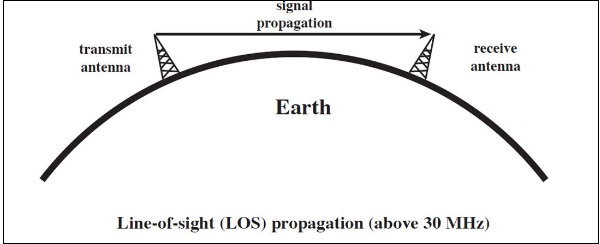The figure depicts this mode of propagation very clearly. The line-of-sight propagation will not be smooth if there occurs any obstacle in its transmission path. As the signal can travel only to lesser distances in this mode, this transmission is used for infrared or microwave transmissions.

## Ground Wave Propagation

Ground wave propagation of the wave follows the contour of earth. Such a wave is called as direct wave. The wave sometimes bends due to the Earth’s magnetic field and gets reflected to the receiver. Such a wave can be termed as reflected wave.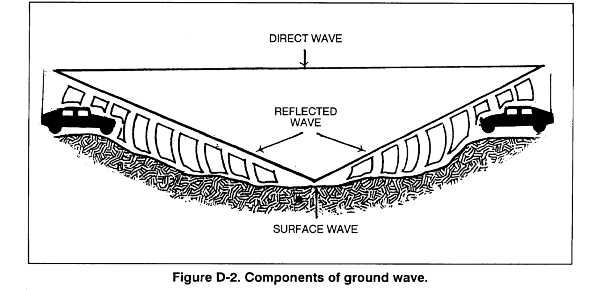The above figure depicts ground wave propagation. The wave when propagates through the Earth’s atmosphere is known as ground wave. The direct wave and reflected wave together contribute the signal at the receiver station. When the wave finally reaches the receiver, the lags are cancelled out. In addition, the signal is filtered to avoid distortion and amplified for clear output.

## Sky Wave Propagation

Sky wave propagation is preferred when the wave has to travel a longer distance. Here the wave is projected onto the sky and it is again reflected back onto the earth.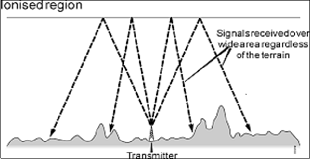The sky wave propagation is well depicted in the above picture. Here the waves are shown to be transmitted from one place and where it is received by many receivers. Hence, it is an example of broadcasting.

The waves, which are transmitted from the transmitter antenna, are reflected from the ionosphere. It consists of several layers of charged particles ranging in altitude from 30- 250 miles above the surface of the earth. Such a travel of the wave from transmitter to the ionosphere and from there to the receiver on Earth is known as Sky Wave Propagation. Ionosphere is the ionized layer around the Earth’s atmosphere, which is suitable for sky wave propagation.

# Antenna Theory – Lonosphere and its Layers

Earth’s atmosphere has several layers. These layers play an important role in the wireless communication. These are mainly classified into three layers.

### Troposphere

This is the layer of the earth, which lies just above the ground. We, the flora and fauna live in this layer. The ground wave propagation and LOS propagation take place here.

### Stratosphere

This is the layer of the earth, which lies above Troposphere. The birds fly in this region. The airplanes travel in this region. Ozone layer is also present in this region. The ground wave propagation and LOS propagation takes place here.

### Ionosphere

This is the upper layer of the Earth’s atmosphere, where ionization is appreciable. The energy radiated by the Sun, not only heats this region, but also produces positive and negative ions. Since the Sun constantly radiates UV rays and air pressure is low, this layer encourages ionization of particles.

## Importance of Ionosphere

The ionosphere layer is a very important consideration in the phase of wave propagation because of the following reasons −

• The layer below ionosphere has higher amount of air particles and lower UV radiation. Due to this, more collisions occur and ionization of particles is minimum and not constant.
• The layer above ionosphere has very low amount of air particles and density of ionization is also quite low. Hence, ionization is not proper.
• The ionosphere has good composition of UV radiation and average air density that does not affect the ionization. Hence, this layer has most influence on the Sky wave propagation.

The ionosphere has different gases with different pressures. Different ionizing agents ionize these at different heights. As various levels of ionization are done at each level, having different gases, few layers with different properties are formed in the ionosphere.

The layers of ionosphere can be studied from the following figure.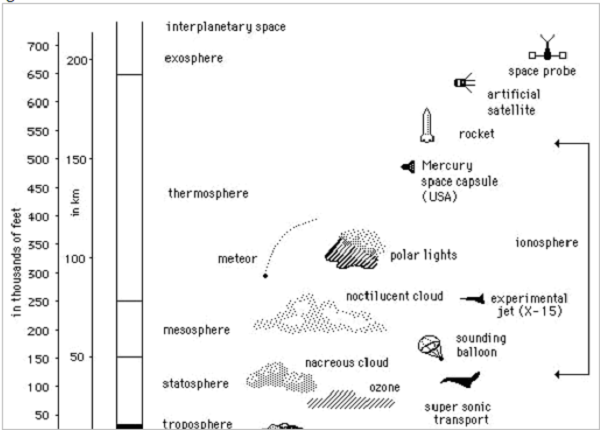The number of layers, their heights, the amount of sky wave that can be bent will vary from day to day, month to month and year to year. For each such layer, there is a frequency, above which if the wave is sent upward vertically, it penetrates through the layer.

The function of these layers depends upon the time of the day, i.e., day time and night time. There are three principal layers- E, F1 and F2 during day time. There is another layer called D layer, which lies below E layer. This layer is at 50 to 90kms above the troposphere.

The following figure depicts the layers present in both day time and night time in the earth’s atmosphere.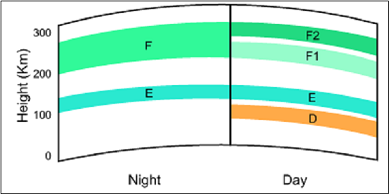This D layer is responsible for the day time attenuation of HF waves. During night time, this D layer almost vanishes out and the F1 and F2 layers combine together to form F layer. Hence, there are only two layers E and F present at the night time.

# Antenna Theory – Terms in Wave Propagation

In the process of propagation of a wave, there are few terms which we come across quite often. Let us discuss about these terms one by one.

## Virtual Height

When a wave is refracted, it is bent down gradually, but not sharply. However, the path of incident wave and reflected wave are same if it is reflected from a surface located at a greater height of this layer. Such a greater height is termed as virtual height.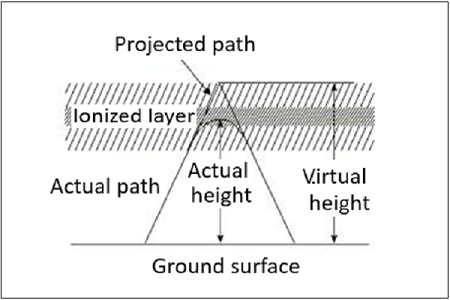The figure clearly distinguishes the virtual height (height of wave, supposed to be reflected) and actual height (the refracted height). If the virtual height is known, the angle of incidence can be found.

## Critical Frequency

Critical frequency for a layer determines the highest frequency that will be returned down to the earth by that layer, after having been beamed by the transmitter, straight up into the sky.

The rate of ionization density, when changed conveniently through the layers, the wave will be bent downwards. The maximum frequency that gets bent and reaches the receiver station with minimum attenuation, can be termed as critical frequency. This is denoted by fc.

## Multi-path

For the frequencies above 30 MHz, the sky wave propagation exists. Signal multipath is the common problem for the propagation of electromagnetic waves going through Sky wave. The wave, which is reflected from the ionosphere, can be called as a hop or skip. There can be a number of hops for the signal as it may move back and forth from the ionosphere and earth surface many times. Such a movement of signal can be termed as multipath.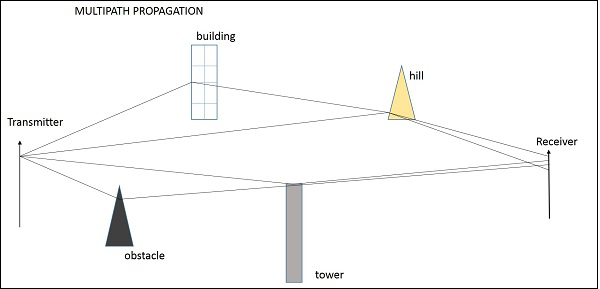The above figure shows an example of multi-path propagation. Multipath propagation is a term, which describes the multiple paths a signal travels to reach the destination. These paths include a number of hops. The paths may be the results of reflection, refraction or even diffraction. Finally, when the signal from such different paths gets to the receiver, it carries propagation delay, additional noise, phase differences etc., which decrease the quality of the received output.

The decrease in the quality of the signal can be termed as fading. This happens because of atmospheric effects or reflections due to multipath.

Fading refers to the variation of the signal strength with respect to time/distance. It is widely prevalent in wireless transmissions. The most common causes of fading in the wireless environment are multipath propagation and mobility (of objects as well as the communicating devices).

## Skip Distance

The measurable distance on the surface of the Earth from transmitter to receiver, where the signal reflected from the ionosphere can reach the receiver with minimum hops or skips, is known as skip distance.

## Maximum Usable Frequency (MUF)

The Maximum Usable Frequency (MUF) is the highest frequency delivered by the transmitter regardless of the power of the transmitter. The highest frequency, which is reflected from the ionosphere to the receiver is called as critical frequency, fc.

MUF=Critical frequencycosθ=fcsecθMUF=Critical frequencycos⁡θ=fcsec⁡θ

## Optimum Working Frequency (OWF)

The frequency, which is being used mostly for a particular transmission and which has been predicted to be used over a particular period of time, over a path, is termed as Optimum Working Frequency (OWF).

## Inter Symbol Interference

Inter symbol interference (ISI) occurs more commonly in communication system. This is the main reason for signal multipath also. When signals arrive at the receiving stations via different propagation paths, they cancel out each other, which is known as the phenomenon of signal fading. Here, it should be remembered that the signals cancel out themselves in vector way.

## Skin Depth

Electromagnetic waves are not suitable for underwater propagations. However, they can propagate under water provided we make the frequency of propagation extremely low. The attenuation of electromagnetic waves under water is expressed in terms of skin depth. Skin depth is defined as the distance at which the signal is attenuated by 1/e. It is a measure of depth to which an EM wave can penetrate. Skin depth is represented as δ (delta).

## Duct Propagation

At a height of around 50 mts from the troposphere, a phenomenon exists; the temperature increases with the height. In this region of troposphere, the higher frequencies or microwave frequencies tend to refract back into the Earth’s atmosphere, instead of shooting into ionosphere, to reflect. These waves propagate around the curvature of the earth even up to a distance of 1000km.

This refraction goes on continuing in this region of troposphere. This can be termed as Super refraction or Duct propagation.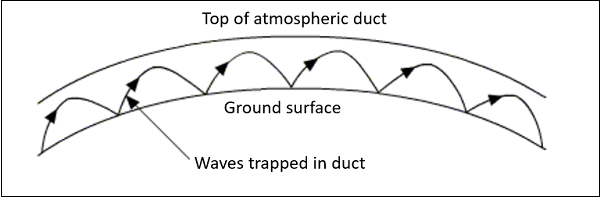The above image shows the process of Duct Propagation. The main requirement for the duct formation is the temperature inversion. The increase of temperature with height, rather than the decrease in the temperature is known as the phenomenon of temperature inversion.

Original Source

Posted on